# JP2011027059A - Engine cotrol apparatus - Google Patents

## Info

Publication number
JP2011027059A
JP2011027059A JP2009175217A JP2009175217A JP2011027059A JP 2011027059 A JP2011027059 A JP 2011027059A JP 2009175217 A JP2009175217 A JP 2009175217A JP 2009175217 A JP2009175217 A JP 2009175217A JP 2011027059 A JP2011027059 A JP 2011027059A
Authority
JP
Japan
Prior art keywords
cylinder
angular acceleration
cyl
air
cylinders
Prior art date
Legal status (The legal status is an assumption and is not a legal conclusion. Google has not performed a legal analysis and makes no representation as to the accuracy of the status listed.)
Pending
Application number
JP2009175217A
Other languages
Japanese (ja)
Inventor
Takanobu Ichihara
Kazuhiko Kanetoshi
Kozo Katogi
Shinji Nakagawa
Minoru Osuga

Original Assignee
Hitachi Automotive Systems Ltd

Priority date (The priority date is an assumption and is not a legal conclusion. Google has not performed a legal analysis and makes no representation as to the accuracy of the date listed.)
Filing date
Publication date
Application filed by Hitachi Automotive Systems Ltd, 日立オートモティブシステムズ株式会社 filed Critical Hitachi Automotive Systems Ltd
Priority to JP2009175217A priority Critical patent/JP2011027059A/en
Publication of JP2011027059A publication Critical patent/JP2011027059A/en
Pending legal-status Critical Current

• 230000001133 acceleration Effects 0.000 claims abstract description 407
• 230000001603 reducing Effects 0.000 claims description 31
• 238000006722 reduction reaction Methods 0.000 claims description 27
• 230000000875 corresponding Effects 0.000 abstract description 6
• 238000000034 methods Methods 0.000 description 8
• 230000000694 effects Effects 0.000 description 4
• 230000001276 controlling effects Effects 0.000 description 2
• 239000000203 mixtures Substances 0.000 description 2
• 239000007789 gases Substances 0.000 description 1

## Images

••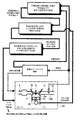•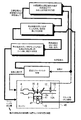•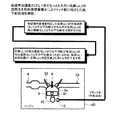•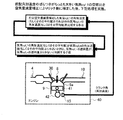•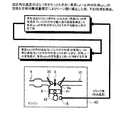•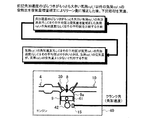•••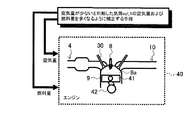•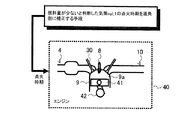•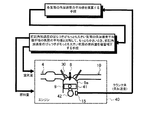•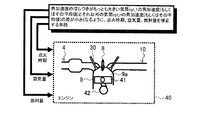•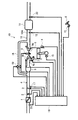•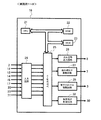•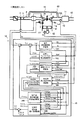•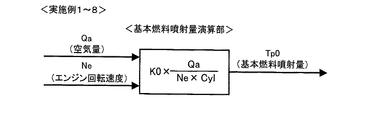•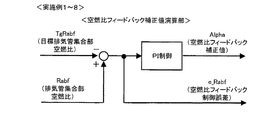•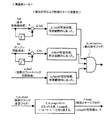•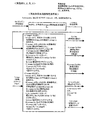••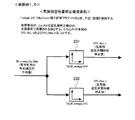•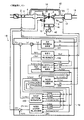•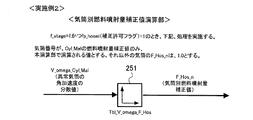•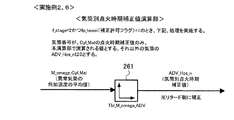•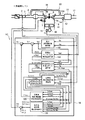••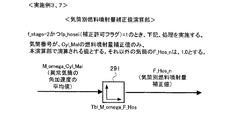•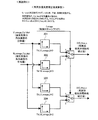•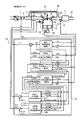•••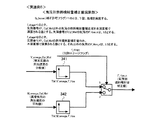•••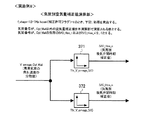## Classifications

• FMECHANICAL ENGINEERING; LIGHTING; HEATING; WEAPONS; BLASTING
• F02COMBUSTION ENGINES; HOT-GAS OR COMBUSTION-PRODUCT ENGINE PLANTS
• F02DCONTROLLING COMBUSTION ENGINES
• F02D41/00Electrical control of supply of combustible mixture or its constituents
• F02D41/008Controlling each cylinder individually
• F02D41/0085Balancing of cylinder outputs, e.g. speed, torque or air-fuel ratio
• FMECHANICAL ENGINEERING; LIGHTING; HEATING; WEAPONS; BLASTING
• F02COMBUSTION ENGINES; HOT-GAS OR COMBUSTION-PRODUCT ENGINE PLANTS
• F02DCONTROLLING COMBUSTION ENGINES
• F02D41/00Electrical control of supply of combustible mixture or its constituents
• F02D41/02Circuit arrangements for generating control signals
• F02D41/14Introducing closed-loop corrections
• F02D41/1497With detection of the mechanical response of the engine
• FMECHANICAL ENGINEERING; LIGHTING; HEATING; WEAPONS; BLASTING
• F02COMBUSTION ENGINES; HOT-GAS OR COMBUSTION-PRODUCT ENGINE PLANTS
• F02DCONTROLLING COMBUSTION ENGINES
• F02D2200/00Input parameters for engine control
• F02D2200/02Input parameters for engine control the parameters being related to the engine
• F02D2200/10Parameters related to the engine output, e.g. engine torque or engine speed
• FMECHANICAL ENGINEERING; LIGHTING; HEATING; WEAPONS; BLASTING
• F02COMBUSTION ENGINES; HOT-GAS OR COMBUSTION-PRODUCT ENGINE PLANTS
• F02DCONTROLLING COMBUSTION ENGINES
• F02D37/00Controlling conjointly two or more functions of engines, not otherwise provided for
• F02D37/02Controlling conjointly two or more functions of engines, not otherwise provided for one of the functions being ignition
• FMECHANICAL ENGINEERING; LIGHTING; HEATING; WEAPONS; BLASTING
• F02COMBUSTION ENGINES; HOT-GAS OR COMBUSTION-PRODUCT ENGINE PLANTS
• F02DCONTROLLING COMBUSTION ENGINES
• F02D41/00Electrical control of supply of combustible mixture or its constituents
• F02D41/02Circuit arrangements for generating control signals
• F02D41/14Introducing closed-loop corrections
• F02D41/1438Introducing closed-loop corrections using means for determining characteristics of the combustion gases; Sensors therefor
• F02D41/1444Introducing closed-loop corrections using means for determining characteristics of the combustion gases; Sensors therefor characterised by the characteristics of the combustion gases
• F02D41/1454Introducing closed-loop corrections using means for determining characteristics of the combustion gases; Sensors therefor characterised by the characteristics of the combustion gases the characteristics being an oxygen content or concentration or the air-fuel ratio

## Abstract

<P>PROBLEM TO BE SOLVED: To correct both the variation in an air-fuel ratio and the torque fluctuation by appropriately performing correction corresponding to an error in a fuel system and an error in an air system. <P>SOLUTION: When a difference between a target air-fuel ratio and the actual air-fuel ratio is less than or equal to a predetermined value during performance of feedback control based on the air-fuel ratio of an exhaust pipe joining portion 10A, for example, the air-fuel ratio of a cylinder cyl_1 having a largest variation in angular acceleration is corrected to a rich side by increasing the amount of fuel. After that, the angular acceleration with respect to each cylinder is detected once again. When the variation in the angular acceleration between the cylinders is not canceled, it is determined that there is an error in the air controlled variable of the cylinder having largest variation. Then, the air volume, fuel quantity, ignition timing and the like of the cylinder are corrected. <P>COPYRIGHT: (C)2011,JPO&INPIT

## Description

The present invention relates to an engine control device, and more particularly to a control device that detects and corrects both fuel amount variation and air amount variation between cylinders.

Against the backdrop of global environmental problems, demands for lower emissions and lower CO 2 (lower fuel consumption) for automobiles are increasing. For the purpose of improving engine performance, engines having a mechanism for independently controlling the amount of fuel and air supplied to the engine, such as the adoption of a variable valve, are becoming widespread. In such an engine, it is necessary to guarantee the performance of independently controlling the fuel amount and the air amount even in a practical environment.

In Patent Document 1, an abnormal cylinder is detected based on rotational fluctuation when a difference between average values of a target air-fuel ratio and an actual air-fuel ratio in a predetermined period is more than a predetermined value, and an empty cylinder is detected based on a fuel injection amount. An invention for correcting the fuel ratio is disclosed.

JP 2004-346807 A

In a mechanism in which the fuel amount and the air amount are controlled independently, the fuel amount and the air amount may vary from cylinder to cylinder. In this case, if control is performed so as to eliminate only the air-fuel ratio variation between the cylinders, the torque between the cylinders varies, and rather the stability (operability) of the engine may deteriorate.

For example, when only the air amount of a specific cylinder increases unintentionally, only the air-fuel ratio of the cylinder becomes lean. If the leaning is corrected by increasing the fuel injection amount of only the cylinder, the torque is increased only for the cylinder and the stability is deteriorated.

The present invention has been made in view of the above points, and an object of the present invention is to control an engine that appropriately corrects both the air-fuel ratio variation and the torque variation according to the cause of the error. To provide an apparatus.

In order to achieve the above object, a first aspect of an engine control apparatus according to the present invention is an engine control apparatus having a plurality of cylinders, and is based on an actual air-fuel ratio of an exhaust pipe collecting portion communicating with each cylinder. Means for performing feedback control of the air-fuel ratio, means for determining that the difference between the target air-fuel ratio and the actual air-fuel ratio is not more than a predetermined value during the air-fuel ratio feedback control, Among the plurality of cylinders, when the difference is determined to be equal to or less than a predetermined value, the means for detecting the cylinder with the largest variation in angular acceleration of the crankshaft, and the air-fuel ratio of the cylinder with the largest variation in angular acceleration And a means for correcting the air-fuel ratio of the cylinders other than the cylinder having the largest variation in angular acceleration to the lean side (see FIG. 1).

In an engine having a plurality of cylinders, when the air-fuel ratio of a certain cylinder becomes lean, when the feedback control based on the air-fuel ratio of the exhaust pipe collecting portion communicating with each cylinder is being performed, the air-fuel ratio of the exhaust pipe collecting portion The fuel injection amount is corrected by the same amount for all the cylinders so that becomes the target air-fuel ratio (generally the theoretical air-fuel ratio).

In many cases, the air-fuel ratio of the exhaust pipe collecting portion is detected after the exhaust gases of all cylinders are sufficiently mixed. This is because, in the operating region where the exhaust flow rate is slow, the exhaust gas is mixed with the exhaust of other cylinders by the exhaust pipe collecting part, and the air-fuel ratio sensor is installed at a position where it is not affected by the sensitivity of a specific cylinder. As a result, it becomes difficult to detect the air-fuel ratio for each cylinder.

As a result, it is common to uniformly correct the fuel injection amounts of all the cylinders so that the average air-fuel ratio of all the cylinders becomes the target air-fuel ratio. Accordingly, when a certain cylinder becomes lean, the average air-fuel ratio of all cylinders also shifts to the lean side, so that the function of uniformly increasing the fuel injection amount of all cylinders automatically works correspondingly.

As a result, the air-fuel ratio of the exhaust pipe collecting portion (average air-fuel ratio of all cylinders) almost converges to the target air-fuel ratio, but the lean cylinder is still lean although the lean degree is small. Other cylinders are rather rich.

On the premise of this operating state, the means described in the first aspect is implemented. That is, as described in the first aspect, during the feedback control based on the air-fuel ratio of the exhaust pipe collecting portion, it is determined that the difference between the target air-fuel ratio and the actual air-fuel ratio is not more than a predetermined value.

If it is determined that the difference between the target air-fuel ratio and the actual air-fuel ratio is less than or equal to a predetermined value (the actual air-fuel ratio converges near the target air-fuel ratio), the variation in angular acceleration correlated with the in-cylinder pressure is It is detected (calculated) separately. A cylinder having a large variation in angular acceleration is determined as a lean cylinder, and the air-fuel ratio of the lean cylinder is corrected to the rich side.

At this time, the air-fuel ratio (average air-fuel ratio of all cylinders) of the exhaust pipe collecting portion temporarily shifts to the rich side due to the effect of correcting the air-fuel ratio of the lean cylinder to the rich side. Since the air-fuel ratio feedback control functions so as to correct to the lean side, the air-fuel ratios of all the cylinders are controlled in the vicinity of the target air-fuel ratio. Alternatively, it is also possible to correct the air-fuel ratio of the cylinders other than the cylinder having a large variation in angular acceleration to the lean side.

At this time, the air-fuel ratio (average air-fuel ratio of all cylinders) of the exhaust pipe collecting portion temporarily shifts to the lean side due to the effect of correcting the air-fuel ratio of the cylinders other than the lean cylinder to the lean side. Since the air-fuel ratio feedback control functions so as to correct the cylinder uniformly to the rich side, the air-fuel ratio of all the cylinders is also controlled in the vicinity of the target air-fuel ratio in this case.

When focusing on a specific cylinder, the air-fuel ratio shift does not necessarily shift to the lean side. However, even if it shifts to the rich side, the other cylinders shift to the lean side by the air-fuel ratio feedback control. Even so, lean cylinders are generated. Further, by frequently performing this control, only the leanest cylinder can be corrected sequentially, and as a result, variations in the air-fuel ratio of all cylinders can be suppressed at all times.

In a second aspect of the engine control apparatus according to the present invention, the means for correcting the air-fuel ratio of the cylinder having the largest variation in angular acceleration to the rich side described in the first aspect is a cylinder having the largest variation in angular acceleration. The fuel amount is corrected to increase or the air amount is corrected to decrease (see FIG. 2). This clearly specifies that the amount of fuel in the cylinder to be corrected is increased or the amount of air is decreased as a method for richly correcting the air-fuel ratio of a cylinder with large variations in angular acceleration.

In a third aspect of the engine control apparatus according to the present invention, the means for correcting the air-fuel ratio of the cylinders other than the cylinder having the largest variation in angular acceleration described in the first aspect to the lean side has the variation in angular acceleration. The fuel amount of the cylinders other than the largest cylinder is corrected to decrease or the air amount is corrected to increase (see FIG. 3). This clearly specifies that the fuel amount of the target cylinder is reduced or the air amount is increased as a method for lean correction of the air-fuel ratio of the cylinders other than the cylinder having a large variation in angular acceleration.

In a fourth aspect of the engine control apparatus according to the present invention, in addition to the above configuration, after correcting the air-fuel ratio of the cylinder cyl_1 having the largest variation in angular acceleration to the rich side by the fuel amount increase correction, the fuel amount increase A means for comparing the corrected angular acceleration of the cylinder cyl_1 or its average value with the angular acceleration of the cylinder cyl_n other than the cylinder cyl_1 having the largest variation in angular acceleration or its average value, and the cylinder cyl_1 having the largest variation in angular acceleration When the angular acceleration of the cylinder cyl_1 other than the cylinder cyl_1 having the largest variation in angular acceleration is larger than the angular acceleration of the cylinder cyl_n or the average value thereof, the amount of air in the cylinder cyl_1 having the largest variation in angular acceleration is Means is provided for determining that the amount of air in the cylinders cyl_n other than the cylinder cyl_1 having the largest variation in angular acceleration is greater than the air amount (FIG. 4). Reference).

That is, in the method described in the first aspect, after correcting the lean cylinder cyl_1 having the largest variation in angular acceleration by increasing the fuel amount, the angular acceleration of the lean cylinder cyl_1 or its average value and the angle of the cylinder cyl_n other than the lean cylinder cyl_1 Compare the acceleration or its average value again.

At this time, if the cause of leaning of the lean cylinder cyl_1 is an unintended increase in air amount, the air-fuel ratio of the lean cylinder cyl_1 is optimized (convergence converged near the target air-fuel ratio) by the fuel amount increase correction, Compared with other cylinders cyl_n other than the lean cylinder cyl_1, the amount of fuel to be supplied increases, so the generated torque increases.

That is, the angular acceleration generated during combustion of the lean cylinder cyl_1 is larger than the angular acceleration generated during combustion of the cylinders cyl_n other than the lean cylinder cyl_1. Therefore, when the angular acceleration of the lean cylinder cyl_1 or its average value is larger than the angular acceleration of the other cylinders cyl_n other than the lean cylinder cyl_1 or its average value, the air amount of the lean cylinder cyl_1 is other cylinders than the lean cylinder cyl_1 It can be determined that there was more air than cyl_n.

In a fifth aspect of the engine control apparatus according to the present invention, in addition to the above configuration, after the air-fuel ratio of the cylinder cyl_1 having the largest variation in angular acceleration is corrected to the rich side by the air amount decrease correction, the air amount decrease A means for comparing the corrected angular acceleration of the cylinder cyl_1 or its average value with the angular acceleration of the cylinder cyl_n other than the cylinder cyl_1 having the largest variation in angular acceleration or its average value, and a cylinder having the largest variation in angular acceleration When the angular acceleration of cyl_1 or the average value thereof is smaller than the angular acceleration of the cylinders cyl_n other than the cylinder cyl_1 having the largest variation in angular acceleration or the average value thereof, the fuel amount of the cylinder cyl_1 having the largest variation in angular acceleration is Means for determining that the amount of fuel in the cylinders cyl_n other than the cylinder cyl_1 having the largest variation in angular acceleration is less than the fuel amount Referring to FIG. 5).

That is, after correcting the lean cylinder cyl_1 by the air amount reduction in the method described in the first aspect, the angular acceleration of the lean cylinder cyl_1 or its average value and the angular acceleration of other cylinders cyl_n other than the lean cylinder cyl_1 or its average value Compare again.

At this time, if the cause of leaning of the lean cylinder cyl_1 is an unintended fuel amount reduction, the air-fuel ratio of the lean cylinder cyl_1 is optimized (converges near the target air-fuel ratio) by the air amount reduction correction, Compared to the cylinders cyl_n other than the lean cylinder cyl_1, the amount of fuel supplied is small, so the torque generated in the lean cylinder cyl_1 is small.

That is, the angular acceleration generated during combustion of the lean cylinder cyl_1 is smaller than the angular acceleration generated during combustion of the cylinders cyl_n other than the lean cylinder cyl_1. Therefore, when the angular acceleration of the lean cylinder cyl_1 or its average value is smaller than the angular acceleration of the other cylinders cyl_n other than the lean cylinder cyl_1 or its average value, the fuel amount of the lean cylinder cyl_1 is other cylinders than the lean cylinder cyl_1 It can be determined that the amount of fuel was less than cyl_n.

In the sixth aspect of the engine control apparatus according to the present invention, in addition to the above configuration, after correcting the air-fuel ratio of the cylinders cyl_n other than the cylinder cyl_1 having the largest variation in angular acceleration to the lean side by the fuel amount reduction correction, Means for comparing the angular acceleration of the cylinder cyl_1 having the largest variation in angular acceleration or an average value thereof with the angular acceleration of the cylinders cyl_n other than the cylinder cyl_1 having the largest variation in acceleration, or the average value thereof; When the angular acceleration of the cylinder cyl_1 having the largest or the average value thereof is larger than the angular acceleration or the average value of the cylinders cyl_n other than the cylinder cyl_1 having the largest variation in the angular acceleration, the variation of the cylinder cyl_1 having the largest variation in the angular acceleration is obtained. The amount of air is larger than the amount of air in the cylinders cyl_n other than the cylinder cyl_1 with the largest variation in angular acceleration. Is provided (see FIG. 6).

That is, after correcting the cylinders cyl_n other than the lean cylinder cyl_1 by the fuel amount reduction in the method described in the first aspect, the angular acceleration of the lean cylinder cyl_1 or its average value and the angular acceleration of the cylinders cyl_n other than the lean cylinder cyl_1 or Compare the average value again.

At this time, if the cause of leaning of the lean cylinder cyl_1 is an unintended increase in the air amount, the fuel amount reduction correction is performed for the cylinders cyl_n other than the lean cylinder cyl_1, so that all cylinders are controlled by air-fuel ratio feedback control. Since the function of uniformly correcting the fuel increase (to the rich side) works, the air-fuel ratio of the lean cylinder cyl_1 is also corrected to the rich side and optimized (convergence is made close to the target air-fuel ratio).

However, as a result, the amount of fuel supplied to the lean cylinder cyl_1 is larger than that of the cylinders cyl_n other than the lean cylinder cyl_1, so that the generated torque increases. That is, the angular acceleration generated during combustion of the lean cylinder cyl_1 is larger than the angular acceleration generated during combustion of the cylinders cyl_n other than the lean cylinder cyl_1.

Therefore, when the angular acceleration of the lean cylinder cyl_1 or its average value is larger than the angular acceleration of the other cylinders cyl_n other than the lean cylinder cyl_1 or its average value, the air amount of the lean cylinder cyl_1 is other cylinders than the lean cylinder cyl_1 It can be determined that there was more air than cyl_n.

In a seventh aspect of the engine control apparatus according to the present invention, in addition to the above configuration, after correcting the air-fuel ratio of the cylinders cyl_n other than the cylinder cyl_1 having the largest variation in angular acceleration to the lean side by the air amount increase correction, Means for comparing the angular acceleration of the cylinder cyl_1 having the largest variation in the angular acceleration or the average value thereof with the angular acceleration of the cylinders cyl_n other than the cylinder cyl_1 having the largest variation in the angular acceleration or the average value thereof; When the angular acceleration of the cylinder cyl_1 having the largest or the average value thereof is smaller than the angular acceleration of the cylinder cyl_n other than the cylinder cyl_1 having the largest variation of the angular acceleration or the average value thereof, the variation of the cylinder cyl_1 having the largest variation of the angular acceleration is obtained. The fuel amount is less than the fuel amount of the cylinders cyl_n other than the cylinder cyl_1 having the largest variation in angular acceleration. Means (see FIG. 7).

That is, after correcting the cylinders cyl_n other than the lean cylinder cyl_1 by the air amount increase in the method described in the first aspect, the angular acceleration of the lean cylinder cyl_1 or an average value thereof and the angular acceleration of the cylinders cyl_n other than the lean cylinder cyl_1 or Compare the average value again.

At this time, if the cause of leaning of the lean cylinder cyl_1 is an unintended fuel amount reduction, air-fuel ratio feedback control is performed by performing an air amount increase correction for the cylinders cyl_n other than the lean cylinder cyl_1 In addition, since the function of correcting the fuel increase uniformly (to the rich side) works for all cylinders, the air-fuel ratio of the lean cylinder cyl_1 is also corrected to the rich side and optimized (convergence is converged to the vicinity of the target air-fuel ratio).

However, since the lean cylinder cyl_1 is still less in amount of supplied fuel than the cylinders cyl_n other than the lean cylinder cyl_1, the generated torque is small. That is, the angular acceleration generated during combustion of the lean cylinder cyl_1 is smaller than the angular acceleration generated during combustion of the cylinders cyl_n other than the lean cylinder cyl_1.

Therefore, when the angular acceleration of the lean cylinder cyl_1 or its average value is smaller than the angular acceleration of the other cylinders cyl_n other than the lean cylinder cyl_1 or its average value, the fuel amount of the lean cylinder cyl_1 is other cylinders than the lean cylinder cyl_1 It can be determined that the amount of fuel was less than cyl_n.

In the eighth aspect of the engine control apparatus according to the present invention, in addition to the configuration of the fourth aspect or the sixth aspect, correction is made so that the air amount and the fuel amount of the cylinder cyl_1 determined to have a large air amount are reduced. Means (see FIG. 8).

That is, in the fourth aspect or the sixth aspect, in order to eliminate the difference between the generated torque of the lean cylinder cyl_1 determined to have a large amount of air and the generated torque of other cylinders cyl_n other than the lean cylinder cyl_1, Correct the air quantity of a certain lean cylinder cyl_1 to be smaller. At this time, the fuel amount is also reduced in accordance with the air amount reduction so that the air-fuel ratio of the lean cylinder cyl_1 does not become rich. As a result, there are no air-fuel ratio and torque variations between the cylinders of the lean cylinder cyl_1 and the cylinders cyl_n other than the lean cylinder cyl_1.

In a ninth aspect of the engine control apparatus according to the present invention, in addition to the configuration of the fourth aspect or the sixth aspect, there is provided means for correcting the ignition timing of the cylinder cyl_1 determined to have a large air amount to the retard side. (See FIG. 9).

That is, in the fourth aspect or the sixth aspect, in order to eliminate the difference between the generated torque of the lean cylinder cyl_1 determined to have a large amount of air and the generated torque of other cylinders cyl_n other than the lean cylinder cyl_1, the lean cylinder cyl_1 The ignition timing is corrected to the retard side. As a result, variations in the air-fuel ratio and torque are eliminated between the lean cylinder cyl_1 and the cylinders cyl_n other than the lean cylinder cyl_1.

In the tenth aspect of the engine control apparatus according to the present invention, in addition to the configuration of the fifth aspect or the seventh aspect, the air amount and the fuel amount of the cylinder cyl_1 determined to be small are corrected so as to increase. Means (see FIG. 10).

That is, in the fifth aspect or the seventh aspect, in order to eliminate the difference between the generated torque of the lean cylinder cyl_1 determined to have a small amount of fuel and the generated torque of other cylinders cyl_n other than the lean cylinder cyl_1, The fuel quantity of a certain lean cylinder cyl_1 is corrected so as to increase. At this time, the air amount is also increased in accordance with the fuel amount increase so that the air-fuel ratio of the lean cylinder cyl_1 does not become rich. As a result, there are no air-fuel ratio and torque variations between the cylinders of the lean cylinder cyl_1 and the cylinders cyl_n other than the lean cylinder cyl_1.

In an eleventh aspect of the engine control apparatus according to the present invention, in addition to the configuration of the fifth aspect or the seventh aspect, means for correcting the ignition timing of the cylinder cyl_1 determined to be small in the fuel amount to an advance side. Provide (see FIG. 11).

That is, in the fifth aspect or the seventh aspect, in order to eliminate the difference between the generated torque of the lean cylinder cyl_1 determined to have a small fuel amount and the generated torque of other cylinders cyl_n other than the lean cylinder cyl_1, the lean cylinder cyl_1 The ignition timing is corrected to the advance side. As a result, variations in the air-fuel ratio and torque are eliminated between the lean cylinder cyl_1 and the cylinders cyl_n other than the lean cylinder cyl_1.

In a twelfth aspect of the engine control apparatus according to the present invention, in addition to the above configuration, the means for calculating the average value of the angular acceleration of each cylinder, the average angular acceleration value of the cylinder having the largest variation in angular acceleration, When comparing the average value of cylinders other than the cylinder with the largest variation in angular acceleration and the average angular acceleration value of the cylinder with the largest variation in angular acceleration being the smallest compared with the average value of other cylinders, Means for increasing the fuel amount of the cylinder having the largest variation in angular acceleration (see FIG. 12).

That is, as described above, a cylinder having a large variation in angular acceleration is determined as a lean cylinder. At the same time, the average value of angular acceleration for each cylinder is calculated. If the engine is leaned due to an unexpected reduction in the fuel amount, the torque of the cylinder is small, and the average angular acceleration value of the cylinder is smaller than the average angular acceleration values of the other cylinders.

On the other hand, if the engine is leaning due to an unexpected increase in air volume, the torque of the cylinder will be small (because the fuel quantity has not decreased), so the average angular acceleration of the cylinder will be Compared to the average value of angular acceleration, it is hardly reduced.

Therefore, when the average angular acceleration value of the cylinder with the largest variation in angular acceleration is the smallest compared to the average value of the other cylinders, the amount of fuel in the cylinder is increased, so that the air-fuel ratio of the cylinder is made lean and Both torque reductions can be solved.

In a thirteenth aspect of the engine control apparatus according to the present invention, in addition to the configurations of the first aspect to the third aspect and the eighth aspect to the eleventh aspect, the angular acceleration of the cylinder cyl_1 having a large variation in angular acceleration or an average thereof Means for correcting the air amount, the fuel amount, and the ignition timing so that the difference between the angular accelerations of the cylinders cyl_n other than the cylinder cyl_1 having the largest variation in the value and the angular acceleration or the average value thereof is reduced (FIG. 13). See).

That is, in the first aspect to the third aspect and the eighth aspect to the eleventh aspect, the generated torque of the cylinder cyl_1 with the large variation in angular acceleration and the generated torque of the cylinders cyl_n other than the cylinder cyl_1 with the largest variation in angular acceleration The difference between the angular acceleration of the cylinder cyl_1 having a large variation in angular acceleration or the average value thereof and the angular acceleration of the cylinder cyl_n other than the cylinder cyl_1 having the largest variation in angular acceleration or the average value thereof is detected. The air amount, the fuel amount, and the ignition timing are corrected so that the difference becomes small (until the difference becomes equal to or less than a predetermined value).

According to the present invention, in a mechanism in which the fuel amount and the air amount are independently controlled, correction is appropriately performed according to each of the fuel system and air system errors, and both the air-fuel ratio variation and the torque variation are corrected. Therefore, even in a practical environment, the engine can be operated stably, so that stable exhaust performance and fuel consumption performance (CO 2 performance) can be realized.

The figure which is provided for description of the 1st aspect of the control apparatus which concerns on this invention. The figure which is provided for description of the 2nd aspect of the control apparatus which concerns on this invention. The figure which is provided for description of the 3rd aspect of the control apparatus which concerns on this invention. The figure which is provided for description of the 4th aspect of the control apparatus which concerns on this invention. The figure which is provided for description of the 5th aspect of the control apparatus which concerns on this invention. The figure which is provided for description of the 6th aspect of the control apparatus which concerns on this invention. The figure which is provided for description of the 7th aspect of the control apparatus which concerns on this invention. The figure which is provided for description of the 8th aspect of the control apparatus which concerns on this invention. The figure which is provided for description of the 9th aspect of the control apparatus which concerns on this invention. The figure which is provided for description of the 10th aspect of the control apparatus which concerns on this invention. The figure which is provided for description of the 11th aspect of the control apparatus which concerns on this invention. The figure which is provided for description of the 12th aspect of the control apparatus which concerns on this invention. The figure which is provided for description of the 13th aspect of the control apparatus which concerns on this invention. The schematic block diagram which shows one Embodiment of the control apparatus which concerns on this invention with the engine to which it is applied. The figure which shows the internal structure of the control unit shown by FIG. The control system figure of Example 1, 5. The figure with which it uses for description of the basic injection fuel amount calculating part of Examples 1-8. The figure which is provided to description of the air fuel ratio feedback correction value calculation part of Examples 1-8. The figure which is provided for description of the detection permission of Examples 1-8 and a control stage calculating part. The figure which is provided for description of the angular acceleration characteristic calculation part according to cylinder of Examples 1, 2, 5, and 6. The figure which is provided for description of the fuel injection amount correction value calculation unit for each cylinder of the first embodiment. The figure which is provided for description of the air amount correction value calculation part for each cylinder of the first and fifth embodiments. The control system figure of Example 2, 6. The figure which is provided for description of the fuel injection amount correction value calculation unit for each cylinder according to the second embodiment. The figure which is provided for description of the ignition timing correction value calculation part according to cylinders of the second and sixth embodiments. FIG. 7 is a control system diagram of the third and seventh embodiments. The figure which is provided for description of the angular acceleration characteristic calculation part according to cylinder of Examples 3, 4, 7, and 8. The figure which is provided for description of the fuel injection amount correction value calculation unit for each cylinder according to the third and seventh embodiments. The figure which is provided for description of the air amount correction value calculation part for each cylinder of the third embodiment. FIG. 9 is a control system diagram of the fourth and eighth embodiments. The figure which is provided for description of the ignition timing correction value calculation unit for each cylinder of the fourth and eighth embodiments. The figure which is provided for description of the air amount correction value calculation part for each cylinder of the fourth embodiment. The figure which is provided for description of the fuel injection amount correction value calculation unit for each cylinder of the fifth embodiment. The figure which is provided for description of the fuel injection amount correction value calculation unit for each cylinder according to the sixth embodiment. FIG. 10 is a diagram which is used for describing a cylinder-by-cylinder air amount correction value calculation unit according to Embodiment 7; FIG. 10 is a diagram which is used for describing a cylinder-by-cylinder air amount correction value calculation unit according to an eighth embodiment.

Hereinafter, embodiments of the present invention will be described with reference to the drawings.
FIG. 14 is a schematic configuration diagram showing an embodiment (common to Examples 1 to 8) of an engine control apparatus according to the present invention, together with an example of an in-vehicle engine to which the engine control device is applied.

The engine 40 is an in-cylinder injection engine composed of a plurality of cylinders 9 (four cylinders in this case). Air from the outside passes through the air cleaner 1, passes through the intake passage 4 and the collector 5, passes through the intake passage 4. It is distributed to the branch passages constituting the downstream portion and flows into the combustion chamber 9a of each cylinder 9.

An intake valve 4 a for opening and closing between the intake passage 4 and the combustion chamber 9 a is disposed in the intake port 4 a at the downstream end portion of the intake passage 4. The amount of inflow air is detected by the airflow sensor 2 and adjusted by the electronic throttle 3 and the intake valve 30.

The intake valve 30 includes a valve mechanism (not shown), is driven based on a drive signal from an intake valve drive circuit 32 of the engine control unit 16, and has a configuration capable of adjusting the lift amount and the opening / closing timing. is doing. The amount of air taken into each cylinder can be adjusted for each cylinder.

The crank angle sensor 15 outputs a signal for each rotation angle 10 ° (deg) of the crankshaft 42 and a signal for each combustion cycle. The intake air temperature sensor 29 detects the intake air temperature, the water temperature sensor 14 detects the engine coolant temperature, and the accelerator opening sensor 13 detects the amount of depression of the accelerator pedal 6, thereby requesting the driver's torque. Is detected.

The signals of the accelerator opening sensor 13, the airflow sensor 2, the intake air temperature sensor 29, the throttle opening sensor 17, the crank angle sensor 15 and the water temperature sensor 14 attached to the electronic throttle 3 are used to control the engine 40 in this embodiment. The engine 40 is sent to an engine control unit (ECU) 16 to obtain the operating state of the engine 40 from the output of each sensor, and the amount of air, the amount of fuel injection, and the main operation amount of the ignition timing are optimally calculated. The

The target air amount calculated in the engine control unit 16 is converted from the target throttle opening to an electronic throttle drive signal and sent to the electronic throttle 3. The fuel injection amount is converted into a valve opening pulse signal and sent to a fuel injection valve (injector) 7. The fuel injection valve 7 is provided in each cylinder 9, and injects fuel into the combustion chamber 9a based on the valve opening pulse signal.

Further, a drive signal is sent to the spark plug 8 so as to be ignited at the ignition timing calculated by the engine control unit 16. The spark plugs 8 are respectively attached so that the ignition parts face the combustion chambers 9a of the cylinders 9.

The fuel injected from the fuel injection valve 7 is mixed with the air from the intake passage 4 to form an air-fuel mixture in the combustion chamber 9a. The air-fuel mixture explodes due to a spark generated from the spark plug 8 at a predetermined ignition timing, and the combustion pressure depresses the piston 41 in the cylinder 9 to serve as power for the engine 40.

Exhaust gas from each cylinder 9 is discharged from the combustion chamber 9a to an individual passage portion forming an upstream portion of the exhaust passage 10 through an exhaust port provided with an exhaust valve, and the exhaust pipe collecting portion 10A is discharged from the individual passage portion. It passes through the three-way catalyst 11 provided in the downstream portion of the exhaust passage 10 and is purified by the three-way catalyst 11 before being discharged to the outside. The three-way catalyst 11 oxidizes hydrocarbons HC and carbon monoxide CO contained in the exhaust gas, and reduces and purifies nitrogen oxides NOx.

A catalyst downstream O 2 sensor 20 is provided downstream of the three-way catalyst 11 in the exhaust passage 10, and an exhaust air-fuel ratio is set in the exhaust pipe assembly 10 A upstream of the catalyst 11 in the exhaust passage 10. A catalyst upstream A / F sensor 12 is provided as an exhaust sensor for detection.

The catalyst upstream A / F sensor 12 has a linear output characteristic with respect to the oxygen concentration contained in the exhaust gas. The relationship between the oxygen concentration in the exhaust gas and the air-fuel ratio is almost linear, and therefore the exhaust air-fuel ratio can be obtained by the catalyst upstream A / F sensor 12 that detects the oxygen concentration.

Then, the engine control unit 16 obtains the exhaust air-fuel ratio upstream of the three-way catalyst 11 from the output signal of the catalyst upstream A / F sensor 12, and oxygen downstream of the three-way catalyst 11 based on the output signal of the catalyst downstream O 2 sensor 20. Determine whether the concentration or stoichiometric is rich or lean.

Also, the fuel injection amount (injected fuel amount) or the air amount is sequentially corrected so that the purification efficiency of the three-way catalyst 11 is optimized using the outputs of the catalyst upstream A / F sensor 12 and the catalyst downstream O 2 sensor 20. B control is performed.

Further, part of the exhaust gas discharged from the combustion chamber 9a to the exhaust passage 10 is returned to the intake passage 4 side through the exhaust recirculation pipe 18 as necessary. The recirculation amount is controlled by an EGR valve 19 provided in the exhaust recirculation pipe 18.

FIG. 15 is an internal configuration diagram of the engine control unit 16. In the ECU 16, there are an airflow sensor 2, a catalyst upstream A / F sensor 12, an accelerator opening sensor 13, a water temperature sensor 14, an engine speed sensor 15, a throttle valve opening sensor 17, a catalyst downstream O 2 sensor 20, an intake air temperature sensor. 29, each sensor output value of the vehicle speed sensor 31 is input, and after signal processing such as noise removal is performed in the input circuit 24, it is sent to the input / output port 25.

The value of the input port is stored in the RAM 23 and processed in the CPU 21. A control program describing the contents of the arithmetic processing is written in the ROM 22 in advance, and values representing each actuator operation amount calculated according to the control program are stored in the RAM 23 and then sent to the input / output port 25.

The operation signal of the spark plug 8 used at the time of spark ignition combustion is set to an ON / OFF signal that is ON when the primary coil in the ignition signal output circuit 26 is energized and is OFF when it is not energized. The ignition timing is when the ignition timing is switched from ON to OFF, and the ignition plug signal set at the output port is amplified by the ignition signal output circuit 26 to a sufficient energy necessary for combustion and supplied to the ignition plug 8.

The drive signal for the fuel injection valve 7 is set to an ON / OFF signal that is ON when the valve is opened and OFF when the valve is closed. The fuel injection valve drive circuit 27 amplifies the drive signal to an energy sufficient to open the fuel injection valve 7. And output to the fuel injection valve 7. Further, a drive signal for realizing the target opening degree of the electronic throttle 3 is output to the electronic throttle 3 through the electronic throttle drive circuit 28. A drive signal for realizing the target lift amount and target opening / closing timing of the intake valve 30 is output to the intake valve 30 via the intake valve drive circuit 32.

Next, a specific example of the control executed by the engine control unit 16 will be described.
[Example 1: Figs. 16 to 22]
FIG. 16 is a control system diagram illustrating the control device 1A according to the first embodiment (fifth embodiment). As shown in the functional block diagram, the engine control unit 16 of the control device 1A includes a basic fuel injection amount calculation unit 161, an air-fuel ratio feedback correction value calculation unit 162, a detection permission and control stage calculation unit 163, and an angle for each cylinder. An acceleration characteristic calculation unit 164, a cylinder specific fuel injection amount correction value calculation unit 165, and a cylinder specific air amount correction value calculation unit 166 are provided. Each of these calculation units is realized by executing a control program in the engine control unit 16.

The basic fuel injection amount calculation unit 161 calculates a basic fuel injection amount Tp0 based on the intake air amount Qa and the engine speed Ne. In the air-fuel ratio feedback correction value calculation unit 162, the fuel injection of all cylinders is performed based on the output (Rabf) of the catalyst upstream A / F sensor 12 so that the exhaust pipe assembly portion air-fuel ratio (Rabf) converges to the target air-fuel ratio. A correction value (Alpha) for correcting the amount equivalently is calculated, and an error (e_Rabf) between the target air-fuel ratio and the exhaust pipe assembly air-fuel ratio is calculated.

The detection permission and control stage calculation unit 163 calculates a detection permission flag (fp_kensyutsu) and a control stage flag (f_stage) for the angular acceleration characteristics for each cylinder in order to perform fuel injection amount correction and air amount correction for each cylinder. . The control stage consists of two stages, stage 1 and stage 2 (details will be described later).

When detection permission and control stage calculation unit 163 receives detection permission (fp_kensyutsu = 1), cylinder specific angular acceleration characteristic calculation unit 164 determines the cylinder number (Cyl_Mal) of the abnormal cylinder that is the cylinder specific angular acceleration characteristic according to each stage. ), The dispersion value (V_omega_Cyl_Mal) of the angular acceleration of the abnormal cylinder, and the average value (M_omega_Cyl_Mal) of the angular acceleration of the abnormal cylinder. Then, after the calculation of the cylinder specific angular acceleration characteristics is completed, a flag (fp_hosei) for permitting correction of the fuel amount and the air amount is calculated.

In the cylinder specific fuel injection amount correction value calculation unit 165, the above-described detection permission and control stage flag (f_stage) obtained by the control stage calculation unit 163, and correction permission flag (fp_hosei) obtained by the cylinder specific angular acceleration characteristic calculation unit 164 Based on the cylinder number (Cyl_Mal) of the abnormal cylinder, the dispersion value (V_omega_Cyl_Mal) of the angular acceleration of the abnormal cylinder, and the average value (M_omega_Cyl_Mal) of the angular acceleration of the abnormal cylinder, the fuel injection amount correction value (F_Hos_n) for each cylinder (n is the cylinder number)).

The cylinder air amount correction value calculation unit 166 then controls the detection permission and control stage flag (f_stage) obtained by the control stage calculation unit 163, and the correction permission flag (fp_hosei) obtained by the cylinder angular acceleration characteristic calculation unit 164. ), The cylinder number (Cyl_Mal) of the abnormal cylinder, and the average value (M_omega_Cyl_Mal) of the angular acceleration of the abnormal cylinder, the air amount correction values (IVO_Hos_n, IVC_Hos_n) for each cylinder are calculated.

Here, IVO_Hos_n is a correction value applied to the intake valve opening timing (IVO_n) of the nth cylinder, and IVC_Hos_n is a correction value applied to the intake valve closing timing (IVC_n) of the nth cylinder. It is. There are various conventional methods for calculating IVO_n and IVC_n, which are not directly related to the present invention and will not be described in detail here.

In the first embodiment, the following processes are controlled.
・ Stage 1 (when f_stage = 1)
(1) The angular acceleration dispersion value is calculated for each cylinder, and the cylinder having the largest angular acceleration dispersion value (the leanest cylinder: the lean cylinder) is detected as an abnormal cylinder (Cyl_Mal). The cylinder number (Cyl_Mal), that is, the dispersion value of the angular acceleration of the abnormal cylinder is defined as V_omega_Cyl_Mal.
(2) On the basis of V_omega_Cyl_Mal, the fuel injection amount is increased and corrected for the abnormal cylinder (F_Hos_n).

・ Stage 2 (when f_stage = 2)
(1) Implemented after stage 1 is completed.
(2) Calculate the average value of angular acceleration for each cylinder, and when the cylinder number with the largest average value of angular acceleration matches the cylinder number (Cyl_Mal) of the abnormal cylinder, the average value of the angular acceleration of the abnormal cylinder is M_omega_Cyl_Mal .
(3) Based on M_omega_Cyl_Mal, the fuel injection amount and the air amount of the abnormal cylinder are reduced and corrected (F_Hos_n, IVO_Hos_n, IVC_Hos_n).
Hereinafter, detailed description of each calculation unit will be given.

<Basic fuel injection amount calculation unit (FIG. 17)>
FIG. 17 is a block diagram showing the function of the basic fuel injection amount calculation unit.

A basic fuel injection amount calculation unit 161 shown in FIG. 16 calculates a basic fuel injection amount (Tp0) based on the intake air amount Qa and the engine speed Ne. Specifically, the calculation is performed by the following equation (1).
Tp0 = K0 × Qa / (Ne × Cyl) (1)

Here, Cyl represents the number of cylinders. K0 is determined based on injector specifications (relationship between fuel injection pulse width and fuel injection amount).

<Air-fuel ratio feedback correction value calculation unit (FIG. 18)>
FIG. 18 is a block diagram showing the function of the air-fuel ratio feedback correction value calculation unit.
An air-fuel ratio feedback correction value calculation unit 162 shown in FIG. 16 calculates a fuel injection amount correction value based on the output (Rabf) of the air-fuel ratio sensor 12. Specifically, as shown in FIG. 18, based on the air-fuel ratio feedback control error (e_Rabf) that is the difference between the target exhaust pipe assembly air-fuel ratio (TgRabf) and the exhaust pipe assembly air-fuel ratio (Rabf), An air-fuel ratio feedback correction value (Alpha) is calculated by PI control. Note that the air-fuel ratio feedback correction value (Alpha) is corrected equivalently to the fuel injection amount of all cylinders.

<Detection permission and control stage calculation unit (FIG. 19)>
FIG. 19 is a block diagram illustrating functions of the detection permission and control stage calculation unit.
The detection permission and control stage calculation unit 163 shown in FIG. 16 calculates a detection permission flag (fp_kensyutsu) and a control stage (f_stage). Specifically, as shown in FIG. 19, the difference (ΔTp0) between the latest basic fuel injection amount (Tp0) and its previous calculated value is calculated, and the latest engine speed (Ne) and its previous calculated value are calculated. The difference (ΔNe) from the above is calculated.

Then, when “ΔTp0 is within a predetermined range for a predetermined time” and “the predetermined time for ΔNe is within a predetermined range” and “the air-fuel ratio feedback control error e_Rabf is within a predetermined range for a predetermined time”, Allow detection of angular acceleration characteristics (fp_kensyutsu = 1).

When the control stage change flag (f_ch_stage) changes from 0 to 1, the value of the control stage flag (f_stage) is switched in order of 1 → 2 → 1 → 2 →. The initial value of the control stage flag (f_stage) is 0, and the first switching is 0 → 1.

<Cylinder-specific angular acceleration characteristic calculation unit (FIG. 20)>
FIG. 20 is a block diagram showing the function of the cylinder specific angular acceleration characteristic calculation unit.
In the cylinder specific angular acceleration characteristic calculation unit 164 shown in FIG. 16, the cylinder number (Cyl_Mal) of the abnormal cylinder, the angular acceleration dispersion value (V_omega_Cyl_Mal) of the abnormal cylinder, the abnormal cylinder, which is the angular acceleration characteristic of each cylinder, according to each stage. The average value of angular acceleration (M_omega_Cyl_Mal) is calculated. Specifically, as shown in FIG. 20, when fp_kensyutsu (detection permission flag) = 1, the following processing is performed.

-Calculate the angular acceleration (omega_n) for each cylinder from the engine speed (Ne). Here, n indicates a cylinder number. An average value of the engine speed Ne is obtained for each combustion cycle, and a difference from the previous average value of the engine speed Ne is defined as an angular acceleration (omega_n).

・ Stage 1 (when f_stage = 1)
(1) From omega_n, the dispersion value (V_omega_n) of omega_n for each cylinder during a predetermined cycle is obtained.
(2) The cylinder number of the cylinder with the largest V_omega_n is Cyl_Mal (abnormal cylinder number), and V_omega_n with the cylinder number Cyl_Mal is V_omega_Cyl_Mal (dispersion value of angular acceleration of the abnormal cylinder).
(3) When fp_kensyutsu = 0 → 1 and the first Cyl_Mal and V_omwga_Cyl_Mal are obtained, fp_hosei = 1.
(4) When V_omega_Cyl_Mal becomes equal to or less than V_omega_n of other cylinders, fp_hosei = 0 and f_ch_stage are set to 1 only once.

・ When stage 2 (when f_stage = 2)
(1) An average value (M_omega_n) of omega_n for each cylinder during a predetermined cycle is obtained from omega_n.
(2) When the cylinder number of the cylinder with the largest M_omega_n is Cyl_Mal (abnormal cylinder number), M_omega_n with the cylinder number Cyl_Mal is defined as M_omega_Cyl_Mal (average value of angular acceleration of the abnormal cylinder).
(3) When fp_kensyutsu = 0 → 1 and the first Cyl_Mal and M_omwga_Cyl_Mal are obtained, fp_hosei (correction permission flag) = 1.
(4) When M_omega_Cyl_Mal becomes equal to or less than M_omega_n of other cylinders, fp_hosei = 0 and f_ch_stage are set to 1 only once.
(5) When fp_kensyutsu = 0, fp_hosei = 0.

<Cylinder-specific fuel injection amount correction value calculation unit (FIG. 21)>
FIG. 21 is a block diagram showing the function of the cylinder specific fuel injection amount correction value calculation unit.
In the cylinder specific fuel injection amount correction value calculation unit 165 shown in FIG. 16, the cylinder specific fuel injection amount correction value (F_Hos_n (n is a cylinder) based on the angular acceleration characteristic obtained by the cylinder specific angular acceleration characteristic calculation unit 164 described above. Number)). Specifically, as shown in FIG. 21, when fp_hosei = 1, the following processing is performed.
Only the fuel injection amount correction value with the cylinder number Cyl_Mal (abnormal cylinder number) is the value calculated by this calculation unit. F_Hos_n (cylinder fuel injection amount correction value) for other cylinders is set to 1.0.
・ In stage 1 (f_stage = 1)
(1) With reference to the table (Tbl_V_omega_F_Hos) 221 from V_omega_Cyl_Mal, F_Hos_n (cylinder-specific fuel injection amount correction value) of the correction target cylinder (cylinder number is Cyl_Mal) is set.
・ When stage 2 (when f_stage = 2)>
(1) With reference to the table (Tbl_M_omega_F_Hos) 222 from M_omega_Cyl_Mal, the correction target cylinder (cylinder number is Cyl_Mal) is set as F_Hos_n (fuel injection amount correction value for each cylinder).

The set value of Tbl_V_omega_F_Hos represents the relationship between the dispersion value of angular acceleration and the air-fuel ratio, and should be determined from the results of actual machine tests. The set value of Tbl_M_omega_F_Hos represents the relationship between the average value of angular acceleration and torque (fuel injection amount corresponding to charging efficiency), and should be determined from the results of actual machine tests. That is, when the average angular acceleration value of the cylinder with the cylinder number Cyl_Mal is larger than the average angular acceleration values of the other cylinders, correction by the cylinder specific fuel injection amount correction value calculation unit 165 is performed.

Since the magnitude of the angular acceleration has a correlation with the magnitude of the torque of the cylinder (abnormal cylinder), the cylinder (abnormal cylinder) of the cylinder (abnormal cylinder) having the cylinder number Cyl_Mal has the same torque as the other cylinders. ) To reduce the amount of fuel. Note that if only the fuel amount is reduced, the cylinder (abnormal cylinder) becomes lean, and therefore the air amount (filling efficiency) of the cylinder (abnormal cylinder) is also reduced by the cylinder-specific air amount correction value calculation unit 166 described later. To do.

<Cylinder-specific air amount correction value calculation unit (FIG. 22)>
FIG. 22 is a block diagram showing functions of the cylinder specific air amount correction value calculation unit.
In the cylinder-specific air amount correction value calculation unit 166 shown in FIG. 16, the cylinder-specific air amount correction values (IVO_Hos_n, IVC_Hos (n is a cylinder) based on the angular acceleration characteristics obtained by the cylinder-specific angular acceleration characteristic calculation unit 164 described above. Number)). Specifically, as shown in FIG. 22, when f_stage = 2 and fp_hosei = 1, the following processing is performed.
(1) Only an air amount correction value with a cylinder number of Cyl_Mal (abnormal cylinder number) is set as a value calculated by this calculation unit. IVO_Hos_n and IVC_Hos_n of other cylinders are set to 0.
(2) With reference to the table (Tbl_M_omega_IVO) 231 from M_omega_Cyl_Mal, the correction target cylinder (cylinder number is Cyl_Mal) is set to IVO_Hos_n.
(3) With reference to the table (Tbl_M_omega_IVC) 232 from M_omega_Cyl_Mal, the correction target cylinder (cylinder number is Cyl_Mal) is set as IVC_Hos_n.

The set values of Tbl_M_omega_IVO_Hos and Tbl_M_omega_IVC_Hos represent the relationship between the average value of angular acceleration and torque (filling efficiency) and should be determined from the results of actual machine tests. That is, when the average angular acceleration value of the cylinder having the cylinder number Cyl_Mal is larger than the average angular acceleration values of the other cylinders, correction by the cylinder air amount correction value calculation unit 166 is performed.

Since the magnitude of the angular acceleration has a correlation with the magnitude of the torque of the cylinder (abnormal cylinder), the torque of the cylinder (Cyl_Mal) with the cylinder number Cyl_Mal is the same as other cylinders (other cylinders other than the abnormal cylinder). Thus, the air amount (filling efficiency) of the cylinder (abnormal cylinder) is reduced. If only the air amount is reduced, the cylinder (abnormal cylinder) becomes rich. Therefore, the fuel amount correction value calculation unit 165 for each cylinder also decreases the fuel amount of the cylinder (abnormal cylinder).

According to the control device 1A of the first embodiment, it is determined that the difference between the target air-fuel ratio and the actual air-fuel ratio is equal to or smaller than a predetermined value during the air-fuel ratio feedback control based on the air-fuel ratio of the exhaust pipe collecting portion. . When it is determined that the difference between the target air-fuel ratio and the actual air-fuel ratio is equal to or less than a predetermined value, it is determined that the actual air-fuel ratio has converged in the vicinity of the target air-fuel ratio, and the angular acceleration correlated with the in-cylinder pressure. Variation (dispersion value, average value) is detected for each cylinder.

Then, among the plurality of cylinders, the cylinder having the largest variation in angular acceleration is identified as an abnormal cylinder (lean cylinder), and the fuel injection amount of the abnormal cylinder is increased and corrected, and the air-fuel ratio of the abnormal cylinder is corrected to the rich side. To do.

At this time, the air-fuel ratio (average air-fuel ratio of all cylinders) of the exhaust pipe collecting portion temporarily shifts to the rich side due to the effect of correcting the air-fuel ratio of the abnormal cylinder to the rich side. Since the air-fuel ratio feedback control functions so as to correct to the lean side, as a result, the air-fuel ratios of all the cylinders are controlled in the vicinity of the target air-fuel ratio.

When focusing on a specific cylinder among the plurality of cylinders, the air-fuel ratio shift does not necessarily shift to the lean side. However, even if the air-fuel ratio shifts to the rich side, the other cylinders are moved to the lean side by air-fuel ratio feedback control. In any case, an abnormal cylinder is generated anyway. Further, by frequently performing this control, only the leanest cylinder can be sequentially corrected as an abnormal cylinder, and as a result, variations in the air-fuel ratio of all cylinders can be constantly suppressed.

Then, after correcting the air-fuel ratio of the abnormal cylinder to the rich side by the fuel amount increase correction, the angular acceleration of the abnormal cylinder corrected by the fuel amount increase or the average value thereof, and the angular acceleration of other cylinders other than the abnormal cylinder or the average value thereof If the angular acceleration of the abnormal cylinder or its average value is greater than the angular acceleration of other cylinders other than the abnormal cylinder or its average value, the amount of air in the abnormal cylinder is Judged to be more than the amount.

If the cause of leaning of the abnormal cylinder is an unintended increase in air amount, the air-fuel ratio of the abnormal cylinder is optimized (convergence converged in the vicinity of the target air-fuel ratio) by correcting the increase in fuel amount. Since the amount of supplied fuel is larger than that of the cylinder, the generated torque is increased. That is, the angular acceleration of the crankshaft that occurs during combustion of the abnormal cylinder is greater than the angular acceleration that occurs during combustion of cylinders other than the abnormal cylinder.

Therefore, when the average value of the angular acceleration of the abnormal cylinder is larger than the average value of the angular acceleration of the cylinders other than the abnormal cylinder, the air amount of the abnormal cylinder is larger than the air amount of the other cylinders other than the abnormal cylinder. It can be judged that there were many.

And it correct | amends so that the air quantity and fuel quantity of an abnormal cylinder judged that there are many air quantities may decrease. In other words, in order to eliminate the difference between the generated torque of the abnormal cylinder determined to have a large amount of air and the generated torque of other cylinders other than the abnormal cylinder, correction is performed so that the amount of air in the abnormal cylinder that is the cause is reduced. To do.

At this time, the fuel amount is also corrected to be small in accordance with the air amount reduction amount so that the air-fuel ratio of the abnormal cylinder does not become rich. As a result, it is possible to eliminate variations in air-fuel ratio and torque between the abnormal cylinder and other cylinders other than the abnormal cylinder.

Further, according to the control device 1A of the first embodiment, an abnormal cylinder is specified and an average value of angular acceleration for each cylinder is calculated. Then, the average angular acceleration value of the abnormal cylinder and the average angular acceleration value of the cylinders other than the abnormal cylinder are compared, and when the average angular acceleration value of the abnormal cylinder is the smallest, the fuel amount of the abnormal cylinder is increased and corrected. .

If the cause of leaning of the abnormal cylinder is an unexpected fuel reduction, the torque of the abnormal cylinder decreases, so the average angular acceleration value of the abnormal cylinder is the same as the average angular acceleration value of other cylinders other than the abnormal cylinder. It becomes small compared.

On the other hand, if the cause of leaning of the abnormal cylinder is an unexpected increase in air volume, the amount of fuel has not decreased, so the torque of the abnormal cylinder is hardly reduced, and the average angular acceleration value of the abnormal cylinder is also other than that of the abnormal cylinder. Compared with the average value of angular acceleration of other cylinders, it is hardly reduced.

Therefore, comparing the average angular acceleration value of the abnormal cylinder with the average value of the cylinders other than the abnormal cylinder, and when the average angular acceleration value of the abnormal cylinder is the smallest, an unexpected fuel loss has occurred. It can be determined, and by increasing the amount of fuel in the abnormal cylinder, both the leaning of the air-fuel ratio and the torque reduction of the abnormal cylinder can be solved.

[Example 2: Figs. 23 to 25]
In the first embodiment described above, when the fuel amount of the abnormal cylinder is increased and corrected, the air-fuel ratio of the abnormal cylinder is corrected to the rich side, and the corrected torque of the abnormal cylinder is larger than the torque of other cylinders other than the abnormal cylinder In the second embodiment, the amount of fuel and air in the abnormal cylinder are corrected to be reduced. In the second embodiment, instead of correcting the amount of fuel and air in the abnormal cylinder to be decreased, the ignition timing of the abnormal cylinder is retarded. It is. That is, in the second embodiment, when the fuel amount of the abnormal cylinder is increased and corrected, the air-fuel ratio of the abnormal cylinder is corrected to the rich side, and the corrected torque of the abnormal cylinder is larger than the torque of other cylinders other than the abnormal cylinder. Is characterized in that the ignition timing of the abnormal cylinder is retarded.

FIG. 23 is a control system diagram illustrating the control device 1B according to the second embodiment.
The engine control unit 16 of the illustrated control device 1B differs from the first embodiment in the specifications of the cylinder specific fuel injection amount correction value calculation unit 165. Further, there is nothing corresponding to the cylinder-by-cylinder air amount correction value calculation unit 166 of the first embodiment, and a difference is that a cylinder-specific ignition timing correction value calculation unit 241 is newly prepared. Since other means are substantially the same as those in the first embodiment, the following description focuses on the parts different from the first embodiment.

The cylinder specific ignition timing correction value calculation unit 241 calculates the cylinder specific ignition timing correction value (ADV_Hos_n) based on the angular acceleration characteristic calculated by the cylinder specific angular acceleration characteristic calculation unit 164 described above. Here, ADV_Hos_n is a correction value applied to the basic ignition timing (ADV0). There are various methods for calculating the basic ignition timing (ADV0) (values that are set so as to optimize fuel consumption under each operating condition), and there are various methods that have not been directly related to the present invention. Do not mention.

In the second embodiment, the following processes are controlled.
・ Stage 1 (when f_stage = 1)
(1) The angular acceleration dispersion value is calculated for each cylinder, and the cylinder having the largest angular acceleration dispersion value (the leanest cylinder: the lean cylinder) is detected as an abnormal cylinder (Cyl_Mal). The dispersion value of the angular acceleration of the cylinder number Cyl_Mal, that is, the abnormal cylinder is V_omega_Cyl_Mal.
(2) On the basis of V_omega_Cyl_Mal, the fuel injection amount is increased and corrected for the abnormal cylinder (F_Hos_n).
・ When stage 2 (when f_stage = 2)
(1) Implemented after stage 1 is completed.
(2) Calculate the average value of angular acceleration for each cylinder, and when the cylinder number with the largest average value of angular acceleration matches the cylinder number (Cyl_Mal) of the abnormal cylinder, the average value of angular acceleration of that cylinder is M_omega_Cyl_Mal (abnormal Average value of the angular acceleration of the cylinder).
(3) Based on M_omega_Cyl_Mal, the ignition timing of the cylinder (abnormal cylinder) is corrected to the retard side (ADV_Hos_n).

Hereinafter, the cylinder specific fuel injection amount correction value calculation unit 165 and the cylinder specific ignition timing correction value calculation unit 241 will be described in detail.

<Cylinder-specific fuel injection amount correction value calculation unit (FIG. 24)>
FIG. 24 is a block diagram showing the function of the cylinder specific fuel injection amount correction value calculator.
In the cylinder specific fuel injection amount correction value calculation unit 165 shown in FIG. 23, the cylinder specific fuel injection amount correction value (F_Hos_n (n is a cylinder) based on the angular acceleration characteristic obtained by the cylinder specific angular acceleration characteristic calculation unit 164 described above. Number)). Specifically, as shown in FIG. 24, when f_stage = 1 and fp_hosei = 1, the following processing is performed.
Only the fuel injection amount correction value with the cylinder number Cyl_Mal (abnormal cylinder number) is the value calculated by this calculation unit. F_Hos_n (cylinder fuel injection amount correction value) for other cylinders is set to 1.0.
Referring to the table (Tbl_V_omega_F_Hos) 251 from V_omega_Cyl_Mal, F_Hos_n (cylinder-specific fuel injection amount correction value) of the correction target cylinder (cylinder number is Cyl_Mal) is set.

The set value of Tbl_V_omega_F_Hos represents the relationship between the dispersion value of angular acceleration and the air-fuel ratio, and should be determined from the results of actual machine tests.

<Ignition Timing Correction Value Calculation Unit for Each Cylinder (FIG. 25)>
FIG. 25 is a block diagram showing the function of the cylinder specific ignition timing correction value calculator.
In the cylinder specific ignition timing correction value calculation unit 241 shown in FIG. 23, the cylinder specific ignition timing correction value (ADV_Hos_n (n is the cylinder number)) based on the angular acceleration characteristic obtained by the cylinder specific angular acceleration characteristic calculation unit 164 described above. ) Is calculated. Specifically, as shown in FIG. 25, when f_stage = 2 and fp_hosei = 1, the following processing is performed.
(1) Only the ignition timing correction value whose cylinder number is Cyl_Mal (abnormal cylinder number) is a value calculated by this calculation unit. ADV_Hos_n (cylinder specific ignition timing correction value) of other cylinders is set to zero.
(2) With reference to the table (Tbl_M_omega_ADV) 261 from M_omega_Cyl_Mal, ADV_Hos_n (cylinder specific ignition timing correction value) of the correction target cylinder (cylinder number is Cyl_Mal) is set.

The set value of Tbl_M_omega_ADV represents the relationship between the average value of angular acceleration and the ignition timing retard amount, and should be determined from the results of actual machine tests.

According to the control device 1B of the second embodiment, the fuel injection amount of the abnormal cylinder is increased and corrected, and the air-fuel ratio of the abnormal cylinder is corrected to the rich side. Then, after correcting the air-fuel ratio of the abnormal cylinder to the rich side by the fuel amount increase correction, the angular acceleration of the abnormal cylinder corrected by the fuel amount increase or the average value thereof, and the angular acceleration of other cylinders other than the abnormal cylinder or the average value thereof If the angular acceleration of the abnormal cylinder or its average value is greater than the angular acceleration of other cylinders other than the abnormal cylinder or its average value, the amount of air in the abnormal cylinder is Judged to be more than the amount. That is, after correcting the abnormal cylinder by increasing the fuel amount, the angular acceleration of the abnormal cylinder or its average value is compared with the angular acceleration of other cylinders other than the abnormal cylinder or its average value.

At this time, if the cause of the leaning of the abnormal cylinder is an unintended increase in the air amount, the air-fuel ratio of the abnormal cylinder is optimized (converges near the target air-fuel ratio) by the fuel amount increase correction, but the abnormal cylinder Compared to other cylinders other than the above, the amount of fuel to be supplied increases, so the generated torque increases. That is, the angular acceleration generated when the abnormal cylinder burns is larger than the angular acceleration generated when the other cylinders other than the abnormal cylinder burn.

Therefore, when the angular acceleration of the abnormal cylinder or its average value is larger than the angular acceleration of other cylinders other than the abnormal cylinder or its average value, the air amount of the abnormal cylinder is larger than the air amount of the other cylinders other than the abnormal cylinder. Can be judged.

Then, the ignition timing of the abnormal cylinder determined to have a large amount of air is corrected to the retard side. That is, the ignition timing of the abnormal cylinder is corrected to the retard side in order to eliminate the difference between the generated torque of the abnormal cylinder determined to have a large amount of air and the generated torque of other cylinders other than the abnormal cylinder. As a result, it is possible to eliminate variations in air-fuel ratio and torque between the abnormal cylinder and other cylinders other than the abnormal cylinder.

[Example 3: Figs. 26 to 29]
In the third embodiment, the air amount of the abnormal cylinder is corrected to be reduced to correct the air-fuel ratio of the abnormal cylinder to the rich side, and when the torque of the corrected abnormal cylinder is smaller than the torque of other cylinders other than the abnormal cylinder, The fuel quantity and air quantity of the abnormal cylinder are increased and corrected.
FIG. 26 is a control system diagram illustrating the control device 1 </ b> C according to the third embodiment.

The third embodiment differs from the first embodiment only in the specifications of the cylinder specific angular acceleration characteristic calculation unit 164, the cylinder specific fuel injection amount correction value calculation unit 165, and the cylinder specific air amount correction value calculation unit 166. Since other means are substantially the same, in the following, calculation units having different specifications will be mainly described.

In the third embodiment, the following processes are controlled.
・ Stage 1 (when f_stage = 1)
(1) The angular acceleration dispersion value is calculated for each cylinder, and the cylinder having the largest angular acceleration dispersion value (the leanest cylinder: the lean cylinder) is detected as an abnormal cylinder (Cyl_Mal). A dispersion value of angular acceleration of a cylinder (cylinder cylinder) with cylinder number Cyl_Mal is V_omega_Cyl_Mal.
(2) Based on V_omega_Cyl_Mal, the air amount is corrected to be reduced for the leanest cylinder (abnormal cylinder) (IVO_Hos_n, IVC_Hos_n).
・ Stage 2 (when f_stage = 2)
(1) Implemented after stage 1 is completed.
(2) Calculate the average value of angular acceleration for each cylinder, and when the cylinder number of the cylinder with the smallest average value of angular acceleration matches Cyl_Mal (cylinder number of abnormal cylinder), the angular acceleration of the cylinder (abnormal cylinder) Let the average value be M_omega_Cyl_Mal (average value of angular acceleration of abnormal cylinders).
(3) Based on M_omega_Cyl_Mal, the fuel injection amount and air amount of the cylinder (abnormal cylinder) are increased and corrected (F_Hos_n, IVO_Hos_n, IVC_Hos_n).

Hereinafter, the cylinder specific angular acceleration characteristic calculation unit 164, the cylinder specific fuel injection amount correction value calculation unit 165, and the cylinder specific air amount correction value calculation unit 166 will be described in detail.

<Cylinder-specific angular acceleration characteristic calculation unit (FIG. 27)>
FIG. 27 is a block diagram showing the function of the cylinder specific angular acceleration characteristic calculating section.
In the cylinder specific angular acceleration characteristic calculation unit 164 shown in FIG. 26, the cylinder number (Cyl_Mal) of the abnormal cylinder, the angular acceleration dispersion value (V_omega_Cyl_Mal), the abnormal cylinder, which is the cylinder specific angular acceleration characteristic, according to each stage. The average value of angular acceleration (M_omega_Cyl_Mal) is calculated.

Specifically, as shown in FIG. 27, when fp_kensyutsu (detection permission flag) = 1, the following processing is performed.
-Calculate the angular acceleration (omega_n) for each cylinder from the engine speed (Ne). Here, n indicates a cylinder number. For the angular acceleration (omega_n), an average value of Ne is obtained for each combustion cycle, and is defined as a difference from the previous Ne.
・ Stage 1 (when f_stage = 1)
(1) From omega_n, the dispersion value (V_omega_n) of omega_n for each cylinder during a predetermined cycle is obtained.
(2) The cylinder number with the largest V_omega_n is Cyl_Mal (abnormal cylinder number), and V_omega_n with the cylinder number Cyl_Mal is V_omega_Cyl_Mal (dispersion value of angular acceleration of the abnormal cylinder).
(3) When fp_kensyutsu = 0 → 1 and the first Cyl_Mal and V_omwga_Cyl_Mal are obtained, fp_hosei (correction permission flag) = 1.
(4) When V_omega_Cyl_Mal becomes equal to or less than V_omega_n of other cylinders, fp_hosei = 0 and f_ch_stage are set to 1 only once.

・ When stage 2 (when f_stage = 2)
(1) An average value (M_omega_n) of omega_n for each cylinder during a predetermined cycle is obtained from omega_n.
(2) When the cylinder number of the cylinder with the smallest M_omega_n is Cyl_Mal (cylinder number of the abnormal cylinder), M_omega_n with the cylinder number Cyl_Mal is set to M_omega_Cyl_Mal (average value of angular acceleration of the abnormal cylinder).
(3) When fp_kensyutsu = 0 → 1 and the first Cyl_Mal and M_omwga_Cyl_Mal are obtained, fp_hosei (correction permission flag) = 1.
(4) When M_omega_Cyl_Mal becomes equal to or greater than M_omega_n of other cylinders, fp_hosei = 0 and f_ch_stage is set to 1 only once.
(5) When fp_kensyutsu = 0, fp_hosei = 0.

<Cylinder-specific fuel injection amount correction value calculation unit (FIG. 28)>
FIG. 28 is a block diagram showing the function of the cylinder specific fuel injection amount correction value calculation unit.
In the cylinder specific fuel injection amount correction value calculation unit 165 shown in FIG. 26, the cylinder specific fuel injection amount correction value (F_Hos_n (n is a cylinder) based on the angular acceleration characteristic obtained by the cylinder specific angular acceleration characteristic calculation unit 164 described above. Number)). Specifically, as shown in FIG. 28, when f_stage = 2 and fp_hosei = 1, the following processing is performed.
Only the fuel injection amount correction value with the cylinder number Cyl_Mal is a value calculated by this calculation unit. F_Hos_n (cylinder fuel injection amount correction value) for other cylinders is set to 1.0.
Referring to the table (Tbl_M_omega_F_Hos) 291 from M_omega_Cyl_Mal, the correction target cylinder (cylinder number is Cyl_Mal) is set as F_Hos_n (fuel injection amount correction value for each cylinder).

The set value of Tbl_M_omega_F_Hos represents the relationship between the average value of angular acceleration and torque (fuel injection amount corresponding to charging efficiency), and should be determined from the results of actual machine tests. That is, when the average angular acceleration value of the cylinder having the cylinder number Cyl_Mal (abnormal cylinder) is smaller than the average angular acceleration values of the other cylinders (other cylinders other than the abnormal cylinder), correction by this calculation unit is performed.

Since the magnitude of the angular acceleration has a correlation with the magnitude of the torque of the cylinder (abnormal cylinder), the torque of the cylinder (Cyl_Mal) with the cylinder number Cyl_Mal is the same as other cylinders (other cylinders other than the abnormal cylinder). Thus, the fuel amount of the cylinder (abnormal cylinder) is increased. Note that when only the fuel amount is increased, the cylinder (abnormal cylinder) becomes rich. Therefore, the air amount correction value calculating unit 166 described later also increases the air amount (filling efficiency) of the cylinder (abnormal cylinder). To do.

<Air amount correction value calculation unit for each cylinder (FIG. 29)>
FIG. 29 is a block diagram illustrating the function of the cylinder-by-cylinder air amount correction value calculation unit 166.
In the cylinder-by-cylinder air amount correction value calculation unit 166 shown in FIG. 26, the cylinder-by-cylinder air amount correction value (IVO_Hos_n, IVC_Hos (n is the cylinder) Number)). Specifically, as shown in FIG. 29, when fp_hosei = 1, the following processing is performed.
-Only the air amount correction value with the cylinder number Cyl_Mal is the value calculated by this calculation unit. IVO_Hos_n and IVC_Hos_n of other cylinders are set to 0.
・ Stage 1 (when f_stage = 1)>
(1) With reference to the table (Tbl_V_omega_IVO) 301 from V_omega_Cyl_Mal, the correction target cylinder (cylinder number is Cyl_Mal) is set to IVO_Hos_n.
(2) Referring to the table (Tbl_V_omega_IVC) 303 from V_omega_Cyl_Mal, the correction target cylinder (cylinder number is Cyl_Mal) is set to IVC_Hos_n.

・ When stage 2 (when f_stage = 2)>
(1) With reference to the table (Tbl_M_omega_IVO) 302 from M_omega_Cyl_Mal, the correction target cylinder (cylinder number is Cyl_Mal) is set to IVO_Hos_n.
(2) With reference to the table (Tbl_M_omega_IVC) 304 from M_omega_Cyl_Mal, IVC_Hos_n of the correction target cylinder (cylinder number is Cyl_Mal) is set.

The set values of Tbl_V_omega_IVO_Hos and Tbl_V_omega_IVC_Hos represent the relationship between the angular acceleration dispersion value and the air-fuel ratio, and should be determined from the results of actual machine tests. The set values of Tbl_M_omega_IVO_Hos and Tbl_M_omega_IVC_Hos represent the relationship between the average value of angular acceleration and the torque (filling efficiency), and are preferably determined from the results of actual machine tests.

When the average angular acceleration value of the cylinder having the cylinder number Cyl_Mal (abnormal cylinder) is smaller than the average angular acceleration value of the other cylinders (cylinders other than the abnormal cylinder), correction is performed by this calculation unit. Since the magnitude of the angular acceleration has a correlation with the magnitude of the torque of the cylinder (abnormal cylinder), the torque of the cylinder (Cyl_Mal) with the cylinder number Cyl_Mal is the same as other cylinders (other cylinders other than the abnormal cylinder). Thus, the air amount (filling efficiency) of the cylinder (abnormal cylinder) is increased. Note that if only the air amount is increased, the cylinder (abnormal cylinder) becomes lean, so that the fuel amount of the abnormal cylinder is also increased in the above-described cylinder-specific fuel amount correction value calculation unit 165.

According to the control device 1C of the third embodiment, the air amount of the abnormal cylinder is corrected to decrease, and the air-fuel ratio of the abnormal cylinder is corrected to the rich side. Then, after correcting the air-fuel ratio of the abnormal cylinder to the rich side by the air amount reduction correction, the angular acceleration of the abnormal cylinder corrected by the air amount reduction or the average value thereof and the angular acceleration of other cylinders other than the abnormal cylinder or the average value thereof are calculated. In comparison, when the angular acceleration of the abnormal cylinder or its average value is smaller than the angular acceleration of the other cylinders other than the abnormal cylinder or its average value, the fuel amount of the abnormal cylinder is greater than the fuel amount of the other cylinders other than the abnormal cylinder. Judge that there are few. That is, after correcting the abnormal cylinder by reducing the air amount, the angular acceleration of the abnormal cylinder or its average value is compared with the angular acceleration of other cylinders other than the abnormal cylinder or its average value.

At this time, if the cause of leaning of the abnormal cylinder is an unintended fuel amount reduction, the air-fuel ratio of the abnormal cylinder is optimized (convergence converged near the target air-fuel ratio) by the air amount reduction correction, but the abnormal cylinder Compared to other cylinders, the amount of fuel supplied is small, so the generated torque is small. That is, the angular acceleration generated when the abnormal cylinder burns is smaller than the angular acceleration generated when the other cylinders other than the abnormal cylinder burn.

Therefore, when the angular acceleration of the abnormal cylinder or the average value thereof is smaller than the angular acceleration of the other cylinders other than the abnormal cylinder or the average value thereof, the fuel amount of the abnormal cylinder is equal to the fuel amount of the other cylinders other than the abnormal cylinder. It can be judged that there was less.

And it correct | amends so that the air quantity and fuel quantity of an abnormal cylinder judged that there are few fuel quantities may be increased. In other words, in order to eliminate the difference between the generated torque of the abnormal cylinder determined to have a small amount of fuel and the generated torque of other cylinders other than the abnormal cylinder, the correction is made to increase the fuel amount of the abnormal cylinder that is the cause. To do.

At this time, the air amount is corrected to be large in accordance with the fuel amount increase so that the air-fuel ratio of the abnormal cylinder does not become rich. As a result, it is possible to eliminate variations in air-fuel ratio and torque between the abnormal cylinder and other cylinders other than the abnormal cylinder.

[Example 4: Figs. 30 to 32]
In the fourth embodiment, the amount of air in the abnormal cylinder is corrected to decrease, the air-fuel ratio of the abnormal cylinder is corrected to the rich side, and the corrected abnormal cylinder torque is smaller than the torque of other cylinders other than the abnormal cylinder, The ignition timing of the abnormal cylinder is corrected to advance.

FIG. 30 is a control system diagram illustrating a control device 1D according to the fourth embodiment.
The fourth embodiment is different from the third embodiment in the specification of the cylinder-by-cylinder air amount correction value calculation unit 166. Further, there is nothing corresponding to the cylinder specific fuel injection amount correction value calculation unit 165 of the third embodiment, and a point that a cylinder specific ignition timing correction value calculation unit 311 is newly prepared is different. Since the other means has substantially the same configuration as that of the third embodiment, the following description focuses on the differences from the third embodiment.

In the fourth embodiment, the following processes are controlled.
・ Stage 1 (f_stage = 1)
(1) The angular acceleration dispersion value is calculated for each cylinder, and the cylinder with the largest angular acceleration dispersion value (the leanest cylinder) is detected as an abnormal cylinder (Cyl_Mal). The cylinder number Cyl_Mal, that is, the dispersion value of the angular acceleration of the abnormal cylinder is V_omega_Cyl_Mal.
(2) Based on V_omega_Cyl_Mal, the air amount is corrected to be reduced for the leanest cylinder (abnormal cylinder) (IVO_Hos_n, IVC_Hos_n).

・ Stage 2 (f_stage = 2)
(1) Implemented after stage 1 is completed.
(2) The average value of angular acceleration is calculated for each cylinder, and when the cylinder number with the smallest average value of angular acceleration matches the cylinder number Cyl_Mal of the abnormal cylinder, the average value of angular acceleration of the cylinder (abnormal cylinder) is calculated as M_omega_Cyl_Mal And
(3) Based on M_omega_Cyl_Mal, the ignition timing of the cylinder (abnormal cylinder) is corrected to the advance side (ADV_Hos_n).

Hereinafter, the cylinder specific ignition timing correction value calculation unit 311 and the cylinder specific air amount correction value calculation unit 166 will be described in detail.

<Ignition Timing Correction Value Calculation Unit for Each Cylinder (FIG. 31)>
FIG. 31 is a block diagram showing the function of the cylinder specific ignition timing correction value calculator.
In the cylinder specific ignition timing correction value calculation unit 311 shown in FIG. 30, the cylinder specific ignition timing correction value (ADV_Hos_n (n is the cylinder number)) based on the angular acceleration characteristic obtained by the cylinder specific angular acceleration characteristic calculation unit 164 described above. ) Is calculated. Specifically, as shown in FIG. 31, when f_stage = 2 and fp_hosei = 1, the following processing is performed.
Only the ignition timing correction value with the cylinder number Cyl_Mal (abnormal cylinder number) is a value calculated by this control unit. ADV_Hos_n of other cylinders is set to zero.
Referring to the table (Tbl_M_omega_ADV) 321 from M_omega_Cyl_Mal, ADV_Hos_n (cylinder-specific ignition timing correction value) of the correction target cylinder (cylinder number is Cyl_Mal) is set. The set value of Tbl_M_omega_ADV represents the relationship between the average value of angular acceleration and the ignition timing advance amount, and should be determined from the results of actual machine tests.

<Air amount correction value calculation unit for each cylinder (FIG. 32)>
FIG. 32 is a block diagram illustrating the function of the cylinder-specific air amount correction value calculation unit.
In the cylinder-specific air amount correction value calculation unit 166 shown in FIG. 30, the cylinder-specific air amount correction values (IVO_Hos_n, IVC_Hos (n is a cylinder) based on the angular acceleration characteristics obtained by the cylinder-specific angular acceleration characteristic calculation unit 164 described above. Number)). Specifically, as shown in FIG. 32, when f_stage = 1 and fp_hosei = 1, the following processing is performed.
(1) Only an air amount correction value with a cylinder number of Cyl_Mal (abnormal cylinder number) is set as a value calculated by this calculation unit. IVO_Hos_n and IVC_Hos_n of other cylinders are set to 0.
(2) With reference to the table (Tbl_V_omega_IVO) 331 from V_omega_Cyl_Mal, the correction target cylinder (cylinder number is Cyl_Mal) is set as IVO_Hos_n.
(3) With reference to the table (Tbl_V_omega_IVC) 332 from V_omega_Cyl_Mal, the correction target cylinder (cylinder number is Cyl_Mal) is set to IVC_Hos_n. The set values of Tbl_V_omega_IVO_Hos and Tbl_V_omega_IVC_Hos represent the relationship between the angular acceleration dispersion value and the air-fuel ratio, and should be determined from the results of actual machine tests.

According to the control device 1D of the fourth embodiment, the air amount of the abnormal cylinder is corrected to decrease, and the air-fuel ratio of the abnormal cylinder is corrected to the rich side. Then, after correcting the air-fuel ratio of the abnormal cylinder to the rich side by the air amount reduction correction, the angular acceleration of the abnormal cylinder corrected by the air amount reduction or the average value thereof and the angular acceleration of other cylinders other than the abnormal cylinder or the average value thereof are calculated. In comparison, when the angular acceleration of the abnormal cylinder or its average value is smaller than the angular acceleration of the other cylinders other than the abnormal cylinder or its average value, the fuel amount of the abnormal cylinder is greater than the fuel amount of the other cylinders other than the abnormal cylinder. Judge that there are few. That is, after correcting the abnormal cylinder by reducing the air amount, the angular acceleration of the abnormal cylinder or its average value is compared with the angular acceleration of other cylinders other than the abnormal cylinder or its average value.

At this time, if the cause of leaning of the abnormal cylinder is an unintended fuel amount reduction, the air-fuel ratio of the abnormal cylinder is optimized (convergence converged near the target air-fuel ratio) by the air amount reduction correction, but the abnormal cylinder Compared to other cylinders, the amount of fuel supplied is small, so the generated torque is small. That is, the angular acceleration generated when the abnormal cylinder burns is smaller than the angular acceleration generated when the other cylinders other than the abnormal cylinder burn.

Therefore, when the angular acceleration of the abnormal cylinder or its average value is smaller than the angular acceleration of other cylinders other than the abnormal cylinder or its average value, the fuel amount of the abnormal cylinder is less than the fuel amount of the other cylinders other than the abnormal cylinder. Can be judged.

Then, the ignition timing of the abnormal cylinder determined to have a small amount of fuel is corrected to the advance side. That is, the ignition timing of the abnormal cylinder is corrected to the advance side in order to eliminate the difference between the generated torque of the abnormal cylinder determined to have a small amount of fuel and the generated torque of other cylinders other than the abnormal cylinder. As a result, it is possible to eliminate variations in air-fuel ratio and torque between the abnormal cylinder and other cylinders other than the abnormal cylinder.

[Example 5: FIG. 33]
In the fifth embodiment, the fuel amount of the cylinders other than the abnormal cylinders is corrected to be reduced, the air-fuel ratio of the cylinders other than the abnormal cylinders is corrected to the lean side, and the torque of the corrected abnormal cylinders other than the abnormal cylinders is corrected. When the torque is larger than the cylinder torque, the amount of fuel and air in the abnormal cylinder is corrected to decrease.

The fifth embodiment is different from the first embodiment only in the specification of the cylinder-by-cylinder fuel injection amount correction value calculation unit 165, and the other means are substantially the same. The calculation unit will be described mainly.

In the fifth embodiment, the following processes are controlled.
・ Stage 1 (f_stage = 1)
(1) The angular acceleration dispersion value is calculated for each cylinder, and the cylinder having the largest angular acceleration dispersion value (the leanest cylinder: the lean cylinder) is detected as an abnormal cylinder (Cyl_Mal). The dispersion value of the angular acceleration of the cylinder number Cyl_Mal, that is, the abnormal cylinder is V_omega_Cyl_Mal.
(2) Based on V_omega_Cyl_Mal, the fuel injection amount is corrected to decrease for the cylinders other than the leanest cylinder (abnormal cylinder) (F_Hos_n).

・ Stage 2 (f_stage = 2)
(1) Implemented after stage 1 is completed.
(2) Calculate the average value of angular acceleration for each cylinder, and when the cylinder number of the cylinder with the largest average value of angular acceleration matches Cyl_Mal (cylinder number of abnormal cylinder), the angular acceleration of the cylinder (abnormal cylinder) Let the average value be M_omega_Cyl_Mal (average value of angular acceleration of abnormal cylinders).
(3) Based on M_omega_Cyl_Mal, the fuel injection amount and air amount of the cylinder (abnormal cylinder) are corrected to decrease (F_Hos_n, IVO_Hos_n, IVC_Hos_n).
Details of the cylinder specific fuel injection amount correction value calculation unit 165 in the fifth embodiment will be described below.

<Cylinder-specific fuel injection amount correction value calculation unit (FIG. 33)>
FIG. 33 is a block diagram illustrating functions of the cylinder specific fuel injection amount correction value calculation unit according to the fifth embodiment. This calculation unit calculates a fuel injection amount correction value (F_Hos_n (n is a cylinder number)) for each cylinder based on the angular acceleration characteristics obtained by the above-described angular acceleration characteristic calculation unit 164 for each cylinder. Specifically, as shown in FIG. 33, when fp_hosei = 1, the following processing is performed.
・ Stage 1 (when f_stage = 1)>
(1) Only the fuel injection amount correction value for cylinders other than the cylinder number Cyl_Mal is a value calculated by this calculation unit. F_Hos_n of the cylinder having the cylinder number Cyl_Mal is set to 1.0.
(2) With reference to the table (Tbl_V_omega_F_Hos) 341 from V_omega_Cyl_Mal, the correction target cylinder (cylinder number other than Cyl_Mal) is set to F_Hos_n.

・ When stage 2 (when f_stage = 2)>
(1) Only the fuel injection amount correction value with a cylinder number of Cyl_Mal is a value calculated by this calculation unit. F_Hos_n of other cylinders is set to 1.0.
(2) With reference to the table (Tbl_M_omega_F_Hos) 342 from M_omega_Cyl_Mal, the correction target cylinder (cylinder number is Cyl_Mal) is set to F_Hos_n.

The set value of Tbl_V_omega_F_Hos represents the relationship between the dispersion value of angular acceleration and the air-fuel ratio, and should be determined from the results of actual machine tests. The set value of Tbl_M_omega_F_Hos represents the relationship between the average value of angular acceleration and torque (fuel injection amount corresponding to charging efficiency), and should be determined from the results of actual machine tests. That is, when the average angular acceleration value of the cylinder with the cylinder number Cyl_Mal is larger than the average angular acceleration values of the other cylinders, correction by this calculation unit is performed. Since the magnitude of the angular acceleration has a correlation with the magnitude of the torque of the cylinder, the amount of fuel in the cylinder is reduced so that the torque of the cylinder having the cylinder number Cyl_Mal is the same as that of the other cylinders.

If only the fuel amount is reduced, the abnormal cylinder becomes lean. Therefore, the air amount (filling efficiency) of the abnormal cylinder is also reduced by the above-described cylinder-by-cylinder air amount correction value calculation unit 166.

According to the control device 1A of the fifth embodiment, the fuel amount of the cylinders other than the abnormal cylinders is corrected to be reduced, and the air-fuel ratio of the cylinders other than the abnormal cylinders is corrected to the lean side.

At this time, the air-fuel ratio (average air-fuel ratio of all cylinders) of the exhaust pipe collecting portion temporarily shifts to the lean side due to the effect of correcting the air-fuel ratio of the cylinders other than the abnormal cylinders to the lean side. Since the air-fuel ratio feedback control functions so as to correct the cylinder uniformly to the rich side, as a result, the air-fuel ratios of all the cylinders are controlled in the vicinity of the target air-fuel ratio.

When focusing on a specific cylinder among the plurality of cylinders, the air-fuel ratio shift does not necessarily shift to the lean side. However, even if the air-fuel ratio shifts to the rich side, the other cylinders are moved to the lean side by air-fuel ratio feedback control. In any case, an abnormal cylinder is generated anyway. Further, by frequently performing this control, only the leanest cylinder can be sequentially corrected as an abnormal cylinder, and as a result, variations in the air-fuel ratio of all cylinders can be constantly suppressed.

Then, after correcting the air-fuel ratio of the cylinders other than the abnormal cylinder to the lean side by the fuel amount reduction correction, the angular acceleration of the abnormal cylinder or the average value thereof, and the angular acceleration of the other cylinders other than the abnormal cylinder or the average value thereof If the angular acceleration of the abnormal cylinder or its average value is greater than the angular acceleration of other cylinders other than the abnormal cylinder or its average value, the amount of air in the abnormal cylinder is Judged to be more than the amount. That is, after correcting cylinders other than the abnormal cylinder by reducing the fuel amount, the angular acceleration of the abnormal cylinder or its average value is compared with the angular acceleration of the other cylinders other than the abnormal cylinder or its average value.

At this time, if the cause of the leaning of the abnormal cylinder is an unintended increase in the air amount, the correction of the fuel amount is reduced for the other cylinders other than the abnormal cylinder so that all cylinders are uniformly controlled by the air-fuel ratio feedback control. Thus, the function of correcting the fuel increase (to the rich side) works, and the air-fuel ratio of the abnormal cylinder is also corrected to the rich side and optimized (convergence is converged to the vicinity of the target air-fuel ratio).

However, as a result, the abnormal cylinder has a larger amount of supplied fuel than the other cylinders than the abnormal cylinder, and therefore the generated torque is increased. That is, the angular acceleration generated when the abnormal cylinder burns is larger than the angular acceleration generated when the other cylinders other than the abnormal cylinder burn.

Therefore, when the angular acceleration of the abnormal cylinder or the average value thereof is larger than the angular acceleration of the other cylinders other than the abnormal cylinder or the average value thereof, the air amount of the abnormal cylinder is larger than the air amount of the other cylinders other than the abnormal cylinder. It can be judged that there were many.

And it correct | amends so that the air quantity and fuel quantity of an abnormal cylinder judged that there are many air quantities may decrease. In other words, in order to eliminate the difference between the generated torque of the abnormal cylinder determined to have a large amount of air and the generated torque of other cylinders other than the abnormal cylinder, correction is performed so that the amount of air in the abnormal cylinder that is the cause is reduced. To do.

At this time, the fuel amount is also corrected to be small in accordance with the air amount reduction amount so that the air-fuel ratio of the abnormal cylinder does not become rich. As a result, it is possible to eliminate variations in air-fuel ratio and torque between the abnormal cylinder and other cylinders other than the abnormal cylinder.

[Example 6: FIG. 34]
In the sixth embodiment, the fuel amount of the cylinders other than the abnormal cylinders is corrected to be decreased, the air-fuel ratio of the cylinders other than the abnormal cylinders is corrected to the lean side, and the torque of the abnormal cylinder after the correction is other than the abnormal cylinders. When the torque is larger than the cylinder torque, the ignition timing of the abnormal cylinder is retarded.

In the sixth embodiment, only the specification of the cylinder specific fuel injection amount correction value calculation unit 165 is different from that of the first embodiment, and the other means are substantially the same. The section will be explained with emphasis.

In Example 6, the following processes are controlled.
・ Stage 1 (f_stage = 1)
(1) The angular acceleration dispersion value is calculated for each cylinder, and the cylinder having the largest angular acceleration dispersion value (the leanest cylinder: the lean cylinder) is detected as an abnormal cylinder (Cyl_Mal). The dispersion value of the angular acceleration of the cylinder number Cyl_Mal, that is, the abnormal cylinder is V_omega_Cyl_Mal.
(2) Based on V_omega_Cyl_Mal, the fuel injection amount is corrected to decrease for the cylinders other than the leanest cylinder (abnormal cylinder) (F_Hos_n).

・ Stage 2 (f_stage = 2)
(1) Implemented after stage 1 is completed.
(2) Calculate the average value of angular acceleration for each cylinder, and when the cylinder number of the cylinder with the largest average value of angular acceleration matches Cyl_Mal (cylinder number of abnormal cylinder), the angular acceleration of the cylinder (abnormal cylinder) Let the average value be M_omega_Cyl_Mal (average value of angular acceleration of abnormal cylinders).
(3) Based on M_omega_Cyl_Mal, the ignition timing of the cylinder (abnormal cylinder) is corrected to the retard side (ADV_Hos_n).
Details of the cylinder specific fuel injection amount correction value calculation unit 165 according to the sixth embodiment will be described below.

<Cylinder-specific fuel injection amount correction value calculation unit (FIG. 34)>
FIG. 34 is a block diagram illustrating functions of the cylinder specific fuel injection amount correction value calculation unit according to the sixth embodiment. This calculation unit calculates a fuel injection amount correction value (F_Hos_n (n is a cylinder number)) for each cylinder based on the angular acceleration characteristics obtained by the above-described angular acceleration characteristic calculation unit 164 for each cylinder. Specifically, as shown in FIG. 34, when f_stage = 1 and fp_hosei = 1, the following processing is performed.
-Only the fuel injection amount correction values for cylinders other than Cyl_Mal are the values calculated by this calculation unit. F_Hos_n of the cylinder having the cylinder number Cyl_Mal is set to 1.0.
Referring to the table (Tbl_V_omega_F_Hos) 351 from V_omega_Cyl_Mal, the correction target cylinder (cylinder number other than Cyl_Mal) is set to F_Hos_n.

The set value of Tbl_V_omega_F_Hos represents the relationship between the dispersion value of angular acceleration and the air-fuel ratio, and should be determined from the results of actual machine tests.

According to the control device 1B of the sixth embodiment, the fuel amount of the cylinders other than the abnormal cylinders is corrected to be reduced, and the air-fuel ratio of the cylinders other than the abnormal cylinders is corrected to the lean side. Then, after correcting the air-fuel ratio of the cylinders other than the abnormal cylinder to the lean side by the fuel amount reduction correction, the angular acceleration of the abnormal cylinder or the average value thereof, and the angular acceleration of the other cylinders other than the abnormal cylinder or the average value thereof If the angular acceleration of the abnormal cylinder or its average value is greater than the angular acceleration of other cylinders other than the abnormal cylinder or its average value, the amount of air in the abnormal cylinder is Judged to be more than the amount. That is, after correcting cylinders other than the abnormal cylinder by reducing the fuel amount, the angular acceleration of the abnormal cylinder or its average value is compared with the angular acceleration of the other cylinders other than the abnormal cylinder or its average value.

At this time, if the cause of the leaning of the abnormal cylinder is an unintended increase in the air amount, the correction of the fuel amount is reduced for the other cylinders other than the abnormal cylinder so that all cylinders are uniformly controlled by the air-fuel ratio feedback control. Thus, the function of correcting the fuel increase (to the rich side) works, and the air-fuel ratio of the abnormal cylinder is also corrected to the rich side and optimized (convergence is converged to the vicinity of the target air-fuel ratio).

However, as a result, the abnormal cylinder has a larger amount of supplied fuel than the other cylinders than the abnormal cylinder, and therefore the generated torque is increased. That is, the angular acceleration generated when the abnormal cylinder burns is larger than the angular acceleration generated when the other cylinders other than the abnormal cylinder burn.

Therefore, when the angular acceleration of the abnormal cylinder or its average value is larger than the angular acceleration of other cylinders other than the abnormal cylinder or its average value, the air amount of the abnormal cylinder is larger than the air amount of the other cylinders other than the abnormal cylinder. Can be judged.

Then, the ignition timing of the abnormal cylinder determined to have a large amount of air is corrected to the retard side. That is, the ignition timing of the abnormal cylinder is corrected to the retard side in order to eliminate the difference between the generated torque of the abnormal cylinder determined to have a large amount of air and the generated torque of other cylinders other than the abnormal cylinder. As a result, it is possible to eliminate variations in air-fuel ratio and torque between the abnormal cylinder and other cylinders other than the abnormal cylinder.

[Example 7: FIG. 35]
In the seventh embodiment, the air amount of other cylinders other than the abnormal cylinders is corrected to be increased to correct the air-fuel ratio of the cylinders other than the abnormal cylinders to the lean side, and the torque of the corrected abnormal cylinders other than the abnormal cylinders is corrected. When the torque is smaller than the torque of the cylinder, the amount of fuel and air in the abnormal cylinder is increased and corrected.

The seventh embodiment is different from the third embodiment described above only in the specification of the cylinder-by-cylinder air amount correction value calculation unit 166, and the other means are substantially the same. The section will be explained with emphasis.

In Example 7, the following processes are controlled.
・ Stage 1 (f_stage = 1)
(1) The angular acceleration dispersion value is calculated for each cylinder, and the cylinder having the largest angular acceleration dispersion value (the leanest cylinder: the lean cylinder) is detected as an abnormal cylinder (Cyl_Mal). The dispersion value of the angular acceleration of the cylinder number Cyl_Mal, that is, the abnormal cylinder is V_omega_Cyl_Mal.
(2) Based on V_omega_Cyl_Mal, the amount of air is corrected to be increased (IVO_Hos_n, IVC_Hos_n) for cylinders other than the leanest cylinder (abnormal cylinder).

・ Stage 2 (f_stage = 2)
(1) Implemented after stage 1 is completed.
(2) The average value of angular acceleration is calculated for each cylinder, and when the cylinder number with the smallest average value of angular acceleration matches the cylinder number Cyl_Mal of the abnormal cylinder, the average value of angular acceleration of the cylinder (abnormal cylinder) is calculated as M_omega_Cyl_Mal (Average value of angular acceleration of abnormal cylinder).
(3) Based on M_omega_Cyl_Mal, the fuel injection amount and air amount of the cylinder (abnormal cylinder) are increased and corrected (F_Hos_n, IVO_Hos_n, IVC_Hos_n).
Details of the cylinder-by-cylinder air amount correction value calculation unit 166 in the seventh embodiment will be described below.

<Air amount correction value calculation unit for each cylinder (FIG. 35)>
FIG. 35 is a block diagram illustrating functions of the cylinder-by-cylinder air amount correction value calculation unit according to the seventh embodiment. This calculation unit calculates the air amount correction values (IVO_Hos_n, IVC_Hos (n is a cylinder number)) for each cylinder based on the angular acceleration characteristics obtained by the above-described angular acceleration characteristic calculation unit 164 for each cylinder. Specifically, as shown in FIG. 35, when fp_hosei = 1, the following processing is performed.

・ Stage 1 (when f_stage = 1)>
(1) Only the air amount correction value of cylinders other than the cylinder number Cyl_Mal is a value calculated by this calculation unit. IVO_Hos_n and IVC_Hos_n of the cylinder having the cylinder number Cyl_Mal are set to 0.
(2) With reference to the table (Tbl_V_omega_IVO) 361 from V_omega_Cyl_Mal, IVO_Hos_n of the correction target cylinder (cylinder number is Cyl_Mal) is set.
(3) With reference to the table (Tbl_V_omega_IVC) 363 from V_omega_Cyl_Mal, the correction target cylinder (cylinder number is Cyl_Mal) is set as IVC_Hos_n.

・ When stage 2 (when f_stage = 2)>
(1) Only the air amount correction value with a cylinder number of Cyl_Mal is set to a value calculated by this calculation unit. IVO_Hos_n and IVC_Hos_n of other cylinders are set to 0.
(2) With reference to the table (Tbl_M_omega_IVO) 362 from M_omega_Cyl_Mal, the correction target cylinder (cylinder number is Cyl_Mal) is set as IVO_Hos_n.
(3) With reference to the table (Tbl_M_omega_IVC) 364 from M_omega_Cyl_Mal, the correction target cylinder (cylinder number is Cyl_Mal) is set to IVC_Hos_n.

The set values of Tbl_V_omega_IVO_Hos and Tbl_V_omega_IVC_Hos represent the relationship between the angular acceleration dispersion value and the air-fuel ratio, and should be determined from the results of actual machine tests. The set values of Tbl_M_omega_IVO_Hos and Tbl_M_omega_IVC_Hos represent the relationship between the average value of angular acceleration and torque (filling efficiency), and should be determined from the results of actual machine tests. That is, when the average angular acceleration value of the cylinder with the cylinder number Cyl_Mal is smaller than the average angular acceleration values of the other cylinders, the correction by this calculation unit is performed.

Since the magnitude of the angular acceleration has a correlation with the magnitude of the torque of the cylinder (abnormal cylinder), the torque of the cylinder (Cyl_Mal) with the cylinder number Cyl_Mal is the same as other cylinders (other cylinders other than the abnormal cylinder). Thus, the air amount (filling efficiency) of the cylinder (abnormal cylinder) is increased. Note that if only the air amount is increased, the cylinder (abnormal cylinder) becomes lean, and therefore the fuel amount of the cylinder (abnormal cylinder) is also increased in the cylinder-specific fuel amount correction value calculation unit 272 described above.

According to the control device 1C of the seventh embodiment, the air amount of the cylinders other than the abnormal cylinders is increased and corrected, and the air-fuel ratio of the cylinders other than the abnormal cylinders is corrected to the lean side. Then, after correcting the air-fuel ratio of the cylinders other than the abnormal cylinder to the lean side by the air amount increase correction, the angular acceleration of the abnormal cylinder or its average value and the angular acceleration of the other cylinders other than the abnormal cylinder or the average value thereof When the angular acceleration of the abnormal cylinder or the average value thereof is smaller than the angular acceleration of the other cylinders other than the abnormal cylinder or the average value thereof, the fuel amount of the abnormal cylinder is the fuel amount of the other cylinders other than the abnormal cylinder. Judge that it is less. That is, after correcting cylinders other than the abnormal cylinder by increasing the air amount, the angular acceleration of the abnormal cylinder or its average value is again compared with the angular acceleration of other cylinders other than the abnormal cylinder or its average value.

At this time, if the cause of leaning of the abnormal cylinder is an unintentional fuel amount decrease, the air amount increase correction is performed on the cylinders other than the abnormal cylinder so that all cylinders are uniformly controlled by air-fuel ratio feedback control. Thus, the function of correcting the fuel increase (to the rich side) works, and the air-fuel ratio of the abnormal cylinder is also corrected to the rich side and optimized (convergence is converged to the vicinity of the target air-fuel ratio).

However, as a result, the abnormal cylinder still has a small amount of supplied fuel compared to other cylinders other than the abnormal cylinder, and thus the generated torque is small. That is, the angular acceleration generated when the abnormal cylinder burns is smaller than the angular acceleration generated when the other cylinders other than the abnormal cylinder burn.

Therefore, when the angular acceleration of the abnormal cylinder or its average value is smaller than the angular acceleration of other cylinders other than the abnormal cylinder or its average value, the fuel amount of the abnormal cylinder is less than the fuel amount of the other cylinders other than the abnormal cylinder. Can be judged.

And it correct | amends so that the air quantity and fuel quantity of an abnormal cylinder judged that there are few fuel quantities may be increased. In other words, in order to eliminate the difference between the generated torque of the abnormal cylinder determined to have a small amount of fuel and the generated torque of other cylinders other than the abnormal cylinder, the correction is made to increase the fuel amount of the abnormal cylinder that is the cause. To do. At this time, the air amount is increased in accordance with the fuel amount increase so that the air-fuel ratio of the abnormal cylinder does not become rich. As a result, it is possible to eliminate variations in the air-fuel ratio and torque between the abnormal cylinder and the cylinders other than the abnormal cylinder.

[Example 8: FIG. 36]
In the eighth embodiment, the air amount of other cylinders other than the abnormal cylinders is corrected to be increased, and the air-fuel ratio of the other cylinders other than the abnormal cylinders is corrected to the lean side. When the torque is smaller than the cylinder torque, the ignition timing of the abnormal cylinder is corrected to advance.

In the eighth embodiment, only the specification of the cylinder-by-cylinder air amount correction value calculation unit 166 is different from that of the fourth embodiment described above, and the other means are substantially the same. Will be explained with emphasis.

In Example 8, the following process is controlled.
・ Stage 1 (f_stage = 1)
(1) The angular acceleration dispersion value is calculated for each cylinder, and the cylinder having the largest angular acceleration dispersion value (the leanest cylinder: the lean cylinder) is detected as an abnormal cylinder (Cyl_Mal). The dispersion value of the angular acceleration of the cylinder number Cyl_Mal, that is, the abnormal cylinder is V_omega_Cyl_Mal.
(2) Based on V_omega_Cyl_Mal, the amount of air is corrected to be increased (IVO_Hos_n, IVC_Hos_n) for cylinders other than the leanest cylinder (abnormal cylinder).

・ Stage 2 (f_stage = 2)
(1) Implemented after stage 1 is completed.
(2) The average value of angular acceleration is calculated for each cylinder, and when the cylinder number with the smallest average value of angular acceleration matches the cylinder number Cyl_Mal of the abnormal cylinder, the average value of angular acceleration of the cylinder (abnormal cylinder) is calculated as M_omega_Cyl_Mal (Average value of angular acceleration of abnormal cylinder).
(3) Based on M_omega_Cyl_Mal, the ignition timing of the cylinder (abnormal cylinder) is corrected to the advance side (ADV_Hos_n).
Details of the cylinder-by-cylinder air amount correction value calculation unit 166 in the eighth embodiment will be described below.

<Air amount correction value calculation unit for each cylinder (FIG. 36)>
FIG. 36 is a block diagram illustrating the function of the cylinder-by-cylinder air amount correction value calculation unit 166 according to the eighth embodiment. This calculation unit calculates the air amount correction values (IVO_Hos_n, IVC_Hos (n is a cylinder number)) for each cylinder based on the angular acceleration characteristics obtained by the above-described angular acceleration characteristic calculation unit 164 for each cylinder. Specifically, as shown in FIG. 36, when f_stage = 1 and fp_hosei = 1, the following processing is performed.
(1) Only the air amount correction value of cylinders other than the cylinder number Cyl_Mal is a value calculated by this calculation unit. IVO_Hos_n and IVC_Hos_n of a cylinder having a cylinder number of Cyl_Mal are set to 0.
(2) With reference to the table (Tbl_V_omega_IVO) 371 from V_omega_Cyl_Mal, the correction target cylinder (cylinder number is Cyl_Mal) is set to IVO_Hos_n.
(3) With reference to the table (Tbl_V_omega_IVC) 372 from V_omega_Cyl_Mal, the correction target cylinder (cylinder number is Cyl_Mal) is set to IVC_Hos_n.

The set values of Tbl_V_omega_IVO_Hos and Tbl_V_omega_IVC_Hos represent the relationship between the angular acceleration dispersion value and the air-fuel ratio, and should be determined from the results of actual machine tests.

According to the control device 1D of the eighth embodiment, the air amount of the cylinders other than the abnormal cylinders is corrected to be increased, and the air-fuel ratio of the cylinders other than the abnormal cylinders is corrected to the lean side. Then, after correcting the air-fuel ratio of the cylinders other than the abnormal cylinder to the lean side by the air amount increase correction, the angular acceleration of the abnormal cylinder or the average value thereof and the angular acceleration of the other cylinders other than the abnormal cylinder or the average value thereof are calculated. In comparison, when the angular acceleration of the abnormal cylinder or its average value is smaller than the angular acceleration of the other cylinders other than the abnormal cylinder or its average value, the fuel amount of the abnormal cylinder is greater than the fuel amount of the other cylinders other than the abnormal cylinder. Judge that there are few. That is, after correcting cylinders other than the abnormal cylinder by increasing the air amount, the angular acceleration of the abnormal cylinder or its average value is compared with the angular acceleration of the other cylinders other than the abnormal cylinder or its average value.

At this time, if the cause of leaning of the abnormal cylinder is an unintentional fuel amount decrease, all the cylinders are controlled by air-fuel ratio feedback control by performing air amount increase correction for other cylinders other than the abnormal cylinder. A function to uniformly increase the fuel amount (to the rich side) works, and the air-fuel ratio of the abnormal cylinder is also corrected to the rich side and optimized (convergence is made close to the target air-fuel ratio).

However, as a result, the abnormal cylinder still has a small amount of supplied fuel compared to other cylinders other than the abnormal cylinder, and thus the generated torque is small. That is, the angular acceleration generated when the abnormal cylinder burns is smaller than the angular acceleration generated when the other cylinders other than the abnormal cylinder burn.

Therefore, when the angular acceleration of the abnormal cylinder or its average value is smaller than the angular acceleration of other cylinders other than the abnormal cylinder or its average value, the fuel amount of the abnormal cylinder is less than the fuel amount of the other cylinders other than the abnormal cylinder. Can be judged.

Then, the ignition timing of the abnormal cylinder determined to have a small amount of fuel is corrected to the advance side. That is, the ignition timing of the abnormal cylinder is corrected to the advance side in order to eliminate the difference between the generated torque of the abnormal cylinder determined to have a small amount of fuel and the generated torque of other cylinders other than the abnormal cylinder. As a result, it is possible to eliminate variations in air-fuel ratio and torque between the abnormal cylinder and other cylinders other than the abnormal cylinder.

DESCRIPTION OF SYMBOLS 1 Air cleaner 2 Air flow sensor 3 Electronic throttle 4 Intake pipe 5 Collector 6 Accelerator 7 Fuel injection valve 8 Spark plug 9 Engine 10 Exhaust pipe 11 Three-way catalyst 12 A / F sensor 13 Accelerator opening sensor 14 Water temperature sensor 15 Engine speed sensor 16 Control unit 17 Throttle opening sensor 18 Exhaust gas recirculation pipe 19 Exhaust gas recirculation amount adjustment valve 20 Catalyst downstream O 2 sensor 21 CPU mounted in control unit
22 ROM mounted in the control unit
23 RAM mounted in the control unit
24 Input circuit 25 of various sensors mounted in the control unit 25 Port for inputting various sensor signals and outputting actuator operation signals 26 Ignition output circuit 27 for outputting drive signals at appropriate timing to the spark plugs Appropriate for fuel injection valves Fuel injection valve drive circuit 28 for outputting a pulse Electronic throttle drive circuit 29 Intake temperature sensor 30 Intake valve 31 Vehicle speed sensor 32 Intake valve drive circuit

## Claims (13)

1. An engine control device having a plurality of cylinders,
Means for performing feedback control of the air-fuel ratio based on the actual air-fuel ratio of the exhaust pipe collecting portion communicating with each cylinder;
Means for determining that the difference between the target air-fuel ratio and the actual air-fuel ratio is not more than a predetermined value during the air-fuel ratio feedback control;
Means for detecting a cylinder having the largest variation in angular acceleration of the crankshaft among the plurality of cylinders when it is determined that the difference between the target air-fuel ratio and the actual air-fuel ratio is not more than a predetermined value;
Means for correcting the air-fuel ratio of the cylinder having the largest variation in angular acceleration to the rich side, or correcting the air-fuel ratio of cylinders other than the cylinder having the largest variation in angular acceleration to the lean side;
An engine control device comprising:
2. Means for correcting the air-fuel ratio of the cylinder with the largest variation in angular acceleration to the rich side,
2. The engine control device according to claim 1, wherein the fuel amount of the cylinder having the largest variation in angular acceleration is corrected to increase or the air amount is corrected to decrease.
3. Means for correcting the air-fuel ratio of cylinders other than the cylinder with the largest variation in angular acceleration to the lean side,
2. The engine control device according to claim 1, wherein the fuel amount of a cylinder other than the cylinder having the largest variation in angular acceleration is corrected to decrease or the amount of air is corrected to increase.
4. After correcting the air-fuel ratio of the cylinder cyl_1 having the largest variation in angular acceleration to the rich side by the fuel amount increase correction,
Means for comparing the angular acceleration of the cylinder cyl_1 corrected with the fuel amount increase or the average value thereof with the angular acceleration of the cylinder cyl_n other than the cylinder cyl_1 having the largest variation in the angular acceleration, or an average value thereof;
The angular acceleration variation of the cylinder cyl_1 having the largest variation in angular acceleration or the average value thereof is compared with the angular acceleration of the cylinder cyl_n other than the cylinder cyl_1 having the largest variation in angular acceleration or the average value thereof. When the angular acceleration of the cylinder cyl_1 having the largest or the average value thereof is larger than the angular acceleration or the average value of the cylinders cyl_n other than the cylinder cyl_1 having the largest variation in the angular acceleration, the variation of the cylinder cyl_1 having the largest variation in the angular acceleration is obtained. The engine control apparatus according to claim 1, further comprising means for determining that the air amount is larger than an air amount of a cylinder cyl_n other than the cylinder cyl_1 having the largest variation in angular acceleration.
5. After correcting the air-fuel ratio of the cylinder cyl_1 having the largest variation in angular acceleration to the rich side by the air amount reduction correction,
Means for comparing the angular acceleration of the cylinder cyl_1 corrected with the air amount reduction or the average value thereof and the angular acceleration of the cylinder cyl_n other than the cylinder cyl_1 having the largest variation in the angular acceleration, or an average value thereof;
When the angular acceleration of the cylinder cyl_1 having the largest variation in angular acceleration or the average value thereof is smaller than the angular acceleration of the cylinders cyl_n other than the cylinder cyl_1 having the largest variation in angular acceleration or the average value thereof, the variation in the angular acceleration is The engine control device according to claim 1, further comprising means for determining that the fuel amount of the largest cylinder cyl_1 is smaller than the fuel amount of the cylinders cyl_n other than the cylinder cyl_1 having the largest variation in angular acceleration. .
6. After correcting the air-fuel ratio of the cylinders cyl_n other than the cylinder cyl_1 having a large variation in angular acceleration to the lean side by the fuel amount reduction correction, the angular acceleration of the cylinder cyl_1 having a large variation in angular acceleration or an average value thereof and the angular acceleration Means for comparing the angular acceleration of the cylinders cyl_n other than the cylinder cyl_1 having the largest variation or the average value thereof;
When the angular acceleration of the cylinder cyl_1 having a large variation in angular acceleration or the average value thereof is larger than the angular acceleration of the cylinder cyl_n other than the cylinder cyl_1 having the largest variation in angular acceleration or the average value thereof, the variation in the angular acceleration is large. The engine control device according to claim 1, further comprising means for determining that an air amount of the cylinder cyl_1 is larger than an air amount of a cylinder cyl_n other than the cylinder cyl_1 having the largest variation in angular acceleration.
7. After correcting the air-fuel ratio of the cylinders cyl_n other than the cylinder cyl_1 having the largest variation in angular acceleration to the lean side by the air amount increase correction,
Means for comparing the angular acceleration of the cylinder cyl_1 having a large variation in angular acceleration or an average value thereof with the angular acceleration of a cylinder cyl_n other than the cylinder cyl_1 having the largest variation in angular acceleration or an average value thereof;
When the angular acceleration of the cylinder cyl_1 having a large variation in angular acceleration or the average value thereof is smaller than the angular acceleration of the cylinders cyl_n other than the cylinder cyl_1 having the largest variation in angular acceleration or the average value thereof, the variation in the angular acceleration is large. The engine control device according to claim 1, further comprising means for determining that the fuel amount of the cylinder cyl_1 is smaller than the fuel amount of the cylinders cyl_n other than the cylinder cyl_1 having the largest variation in angular acceleration.
8.   The engine control device according to claim 4 or 6, further comprising means for correcting the air amount and the fuel amount of the cylinder cyl_1 determined to have a large air amount.
9.   The engine control device according to claim 4 or 6, further comprising means for correcting the ignition timing of the cylinder cyl_1 determined to have a large amount of air to the retard side.
10.   The engine control device according to claim 5 or 7, further comprising means for correcting the air amount and the fuel amount of the cylinder cyl_1 determined to have a small fuel amount.
11.   The engine control device according to claim 5 or 7, further comprising means for correcting an ignition timing of the cylinder cyl_1 determined to be low in fuel amount to an advance side.
12. Means for calculating an average value of angular acceleration of each cylinder;
Comparing the average angular acceleration value of the cylinder with the largest variation in angular acceleration with the average value of the cylinders other than the cylinder having the largest variation in angular acceleration, the average angular acceleration value of the cylinder with the largest variation in angular acceleration 2. The engine control device according to claim 1, further comprising means for correcting the amount of fuel in the cylinder having the largest variation in angular acceleration when the difference is the smallest.
13. The amount of air so that the difference between the angular acceleration of the cylinder cyl_1 having a large variation in angular acceleration or the average value thereof and the angular acceleration of the cylinders cyl_n other than the cylinder cyl_1 having the largest variation in angular acceleration or the average value thereof is reduced. 13. The engine control apparatus according to claim 1, further comprising means for correcting the fuel amount and the ignition timing.
JP2009175217A 2009-07-28 2009-07-28 Engine cotrol apparatus Pending JP2011027059A (en)

## Priority Applications (1)

Application Number Priority Date Filing Date Title
JP2009175217A JP2011027059A (en) 2009-07-28 2009-07-28 Engine cotrol apparatus

## Applications Claiming Priority (3)

Application Number Priority Date Filing Date Title
JP2009175217A JP2011027059A (en) 2009-07-28 2009-07-28 Engine cotrol apparatus
US12/826,367 US8267076B2 (en) 2009-07-28 2010-06-29 Engine control apparatus
EP10167653.4A EP2284378B1 (en) 2009-07-28 2010-06-29 Engine control apparatus

## Publications (1)

Publication Number Publication Date
JP2011027059A true JP2011027059A (en) 2011-02-10

# Family

## Family Applications (1)

Application Number Title Priority Date Filing Date
JP2009175217A Pending JP2011027059A (en) 2009-07-28 2009-07-28 Engine cotrol apparatus

## Country Status (3)

US (1) US8267076B2 (en)
EP (1) EP2284378B1 (en)
JP (1) JP2011027059A (en)

## Cited By (4)

* Cited by examiner, † Cited by third party
Publication number Priority date Publication date Assignee Title
WO2012153403A1 (en) * 2011-05-11 2012-11-15 トヨタ自動車株式会社 Control device for internal combustion engine
JP2013096354A (en) * 2011-11-04 2013-05-20 Toyota Motor Corp Internal combustion engine control device
JP2013217295A (en) * 2012-04-09 2013-10-24 Hitachi Automotive Systems Ltd Engine control device
WO2014112427A1 (en) * 2013-01-18 2014-07-24 日立オートモティブシステムズ株式会社 Control device and control method of engine

## Families Citing this family (14)

* Cited by examiner, † Cited by third party
Publication number Priority date Publication date Assignee Title
JP5103459B2 (en) * 2009-10-30 2012-12-19 日立オートモティブシステムズ株式会社 Engine control device
JP5111529B2 (en) * 2010-01-22 2013-01-09 日立オートモティブシステムズ株式会社 Control diagnosis device for internal combustion engine
DE102010051034A1 (en) * 2010-11-11 2012-05-16 Daimler Ag Method for determining a type of air-fuel mixture error
JP5962171B2 (en) * 2012-04-24 2016-08-03 スズキ株式会社 Combustion state control device for internal combustion engine of vehicle
JP5844218B2 (en) * 2012-05-29 2016-01-13 愛三工業株式会社 Control device for internal combustion engine
JP6160035B2 (en) * 2012-07-05 2017-07-12 トヨタ自動車株式会社 Cylinder air-fuel ratio variation abnormality detecting device for multi-cylinder internal combustion engine
JP5941077B2 (en) * 2014-01-31 2016-06-29 富士重工業株式会社 Fuel injection device
EP2907993B1 (en) * 2014-02-13 2019-11-06 Caterpillar Motoren GmbH & Co. Method for balancing cylinders of an internal combustion engine
JP6286236B2 (en) * 2014-03-10 2018-02-28 日野自動車株式会社 Engine system abnormality determination device
WO2015136087A1 (en) * 2014-03-13 2015-09-17 Husqvarna Ab Method for optimizing a/f ratio during acceleration and a hand held machine
JP6462311B2 (en) * 2014-10-24 2019-01-30 日立オートモティブシステムズ株式会社 Engine control device
US9759148B2 (en) * 2015-05-14 2017-09-12 Ford Global Technologies, Llc Method and system for determining air-fuel ratio imbalance via engine torque
DE102016208195A1 (en) * 2016-05-12 2017-11-16 Robert Bosch Gmbh Method for fault diagnosis in an internal combustion engine
CN107975434A (en) * 2017-11-16 2018-05-01 中国第汽车股份有限公司 Each cylinder air-fuel ratio inhomogeneities detection method of direct spray petrol engine in cylinder and system

## Citations (1)

* Cited by examiner, † Cited by third party
Publication number Priority date Publication date Assignee Title
JP2000205025A (en) * 1999-01-14 2000-07-25 Fuji Heavy Ind Ltd Control apparatus for engine

## Family Cites Families (9)

* Cited by examiner, † Cited by third party
Publication number Priority date Publication date Assignee Title
JPS5851243A (en) * 1981-09-24 1983-03-25 Nippon Denso Co Ltd Method of detecting combustion condition of internal- combustion engine
JP3085382B2 (en) * 1989-08-25 2000-09-04 株式会社日立製作所 Method for controlling combustion state of internal combustion engine
JP2907001B2 (en) * 1994-05-26 1999-06-21 三菱自動車工業株式会社 Lean combustion control and failure determination device for internal combustion engine
US6382198B1 (en) * 2000-02-04 2002-05-07 Delphi Technologies, Inc. Individual cylinder air/fuel ratio control based on a single exhaust gas sensor
JP4296838B2 (en) 2003-05-21 2009-07-15 株式会社デンソー Engine control device
DE102005014920A1 (en) * 2005-04-01 2006-04-13 Audi Ag Method to adjust injection times of individual cylinders of an internal combustion engine depending on their dimensions derived from the crank shaft rotations to compensate for fluctuations in injection quantities
US7047957B1 (en) * 2005-04-25 2006-05-23 Delphi Technologies, Inc. Method and apparatus for monitoring a multiple step valve lifter
US7497210B2 (en) * 2006-04-13 2009-03-03 Denso Corporation Air-fuel ratio detection apparatus of internal combustion engine
JP5103459B2 (en) * 2009-10-30 2012-12-19 日立オートモティブシステムズ株式会社 Engine control device

## Patent Citations (1)

* Cited by examiner, † Cited by third party
Publication number Priority date Publication date Assignee Title
JP2000205025A (en) * 1999-01-14 2000-07-25 Fuji Heavy Ind Ltd Control apparatus for engine

## Cited By (9)

* Cited by examiner, † Cited by third party
Publication number Priority date Publication date Assignee Title
WO2012153403A1 (en) * 2011-05-11 2012-11-15 トヨタ自動車株式会社 Control device for internal combustion engine
JP5083583B1 (en) * 2011-05-11 2012-11-28 トヨタ自動車株式会社 Control device for internal combustion engine
US20140058646A1 (en) * 2011-05-11 2014-02-27 Toyota Jidosha Kabushiki Kaisha Control device of an engine
US9194322B2 (en) 2011-05-11 2015-11-24 Toyota Jidosha Kabushiki Kaisha Control device of an engine
JP2013096354A (en) * 2011-11-04 2013-05-20 Toyota Motor Corp Internal combustion engine control device
JP2013217295A (en) * 2012-04-09 2013-10-24 Hitachi Automotive Systems Ltd Engine control device
WO2014112427A1 (en) * 2013-01-18 2014-07-24 日立オートモティブシステムズ株式会社 Control device and control method of engine
JP2014137041A (en) * 2013-01-18 2014-07-28 Hitachi Automotive Systems Ltd Device and method for controlling engine
US10012160B2 (en) 2013-01-18 2018-07-03 Hitachi Automotive Systems, Ltd. Control device and control method of engine

## Also Published As

Publication number Publication date
US8267076B2 (en) 2012-09-18
EP2284378B1 (en) 2016-07-13
EP2284378A2 (en) 2011-02-16
EP2284378A3 (en) 2014-06-04
US20110029218A1 (en) 2011-02-03

## Similar Documents

Publication Publication Date Title
US6928983B2 (en) Fuel injection controller for internal combustion engine
JP4276241B2 (en) Engine control device
JP4251073B2 (en) Control device for internal combustion engine
JP4243598B2 (en) Control device for internal combustion engine
JP4226580B2 (en) Control device for internal combustion engine
US6644017B2 (en) Device for and method of controlling air-fuel ratio of internal combustion engine
JP4363398B2 (en) Air-fuel ratio control device for internal combustion engine
JP4315196B2 (en) Control device for internal combustion engine
JP4178386B2 (en) Control device for knocking suppression of internal combustion engine
US7013873B2 (en) Apparatus and method for controlling fuel injection in internal combustion engine
US20070215095A1 (en) Controller for compression ignition engine
JP4130800B2 (en) Engine control device
JP4192759B2 (en) Injection quantity control device for diesel engine
JP5348190B2 (en) Control device for internal combustion engine
JP4065784B2 (en) Control device for internal combustion engine
JP5103459B2 (en) Engine control device
JP3984439B2 (en) Control device for internal combustion engine
JP4277897B2 (en) Control device for internal combustion engine
US20090017987A1 (en) Control Method and Control Device for Engine
JP2005048730A (en) Air fuel ratio control device of internal combustion engine
US9043121B2 (en) Air-fuel ratio variation abnormality detecting device and air-fuel ratio variation abnormality detecting method
JP2006336503A (en) Air-fuel ratio control device for internal combustion engine
EP2284378B1 (en) Engine control apparatus
US6237329B1 (en) Combustion controller for lean burn engines
JP5858159B2 (en) Internal combustion engine

## Legal Events

Date Code Title Description
A621 Written request for application examination

Free format text: JAPANESE INTERMEDIATE CODE: A621

Effective date: 20110701

A977 Report on retrieval

Free format text: JAPANESE INTERMEDIATE CODE: A971007

Effective date: 20111027

A131 Notification of reasons for refusal

Free format text: JAPANESE INTERMEDIATE CODE: A131

Effective date: 20111101

A521 Written amendment

Free format text: JAPANESE INTERMEDIATE CODE: A523

Effective date: 20111226

A02 Decision of refusal

Free format text: JAPANESE INTERMEDIATE CODE: A02

Effective date: 20120306